knitr::opts_chunk$set(fig.retina=2) library(dplyr) library(tidyr) library(purrr) library(ggplot2) library(ggstance) library(stringr) library(magrittr) library(DT) library(scales) library(countrycode) library(tm) source(file.path(PROJHOME, "Analysis", "lib", "graphic_functions.R")) # Load cleaned, country-based survey data (*with* the Q4\* loop) survey.clean.all <- readRDS(file.path(PROJHOME, "Data", "data_processed", "survey_clean_all.rds")) # Load cleaned, organization-based data (without the Q4 loop) survey.orgs.clean <- readRDS(file.path(PROJHOME, "Data", "data_processed", "survey_orgs_clean.rds")) # Load cleaned, country-based data (only the Q4 loop) survey.countries.clean <- readRDS(file.path(PROJHOME, "Data", "data_processed", "survey_countries_clean.rds")) # Load Robinson map projection countries.ggmap <- readRDS(file.path(PROJHOME, "Data", "data_processed", "countries110_robinson_ggmap.rds")) # All possible countries (to fix the South Sudan issue) possible.countries <- data_frame(id = unique(as.character(countries.ggmap$id)))

## General questions

### How many NGOs responded?

nrow(survey.orgs.clean) 
##  641

### How many respondents answered questions for more than one country?

survey.countries.clean %>% filter(loop.number > 1) %>% nrow %T>%
{print(percent(. / nrow(survey.orgs.clean)))}
##  "2.81%"
##  18

### Who in the organization responded to the survey?

df.plot.respondents <- survey.orgs.clean %>%
group_by(Q2.3) %>%
summarise(num = n()) %>%
arrange(num) %>%
mutate(question = factor(Q2.3, levels=Q2.3, ordered=TRUE))

plot.respondents <- ggplot(df.plot.respondents, aes(x=num, y=question)) +
geom_barh(stat="identity") +
scale_x_continuous(expand=c(0, 0)) +
labs(x="Number of respondents", y=NULL,
title="Who filled out the survey?",
theme_ath()

plot.respondents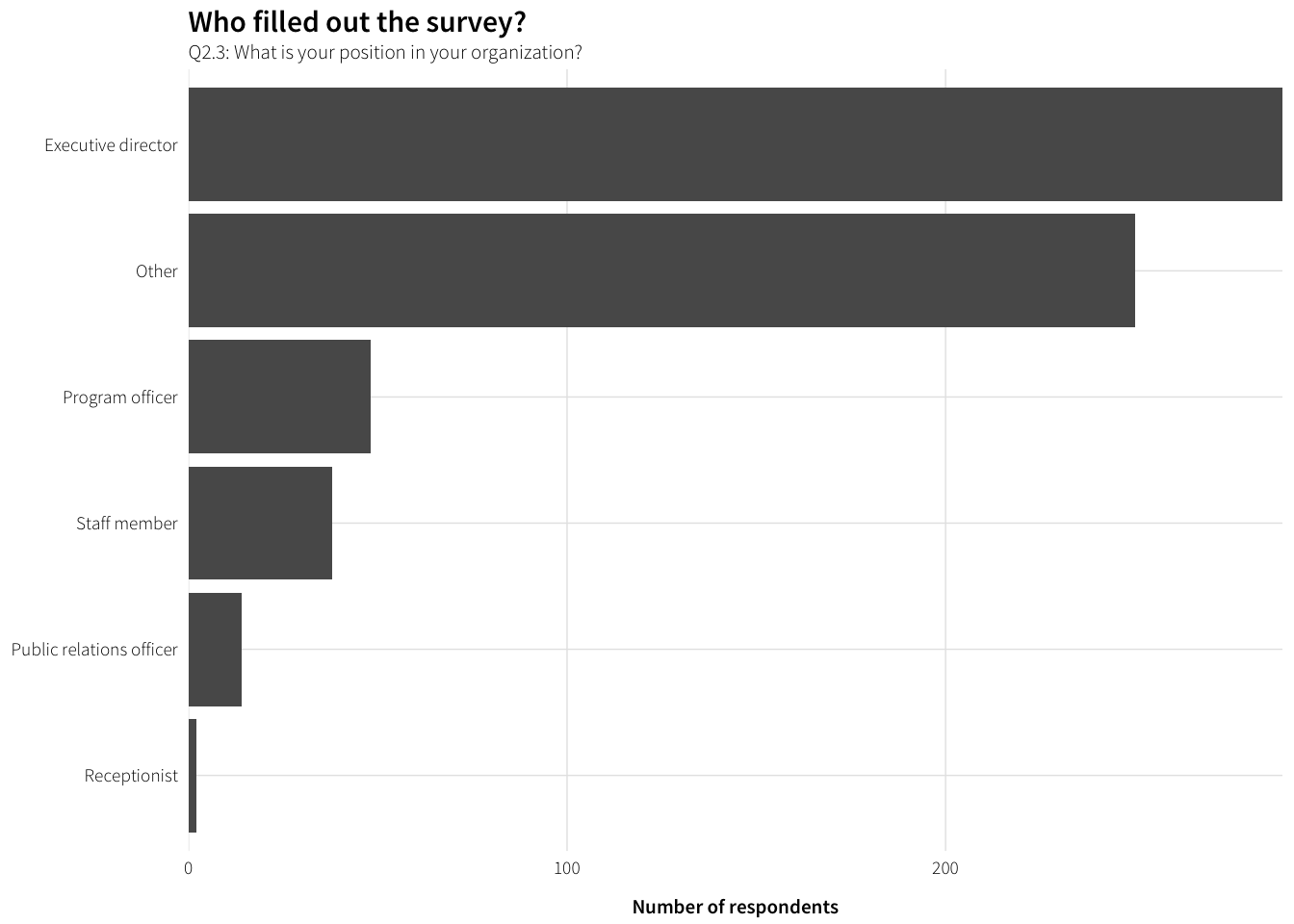Lots of others. Who are the others?

# Table
position.in.org <- survey.orgs.clean %>%
filter(!is.na(Q2.3_TEXT)) %>%
mutate(position.in.org = str_to_title(Q2.3_TEXT)) %>%
group_by(position.in.org) %>%
summarise(num = n()) %>%
arrange(desc(num))

datatable(position.in.org)
# Figure
df.plot.respondents.other <- position.in.org %>%
filter(num >= 5) %>%
mutate(question = factor(position.in.org,
levels=rev(position.in.org), ordered=TRUE))

plot.respondents.other <- ggplot(df.plot.respondents.other,
aes(x=num, y=question)) +
geom_barh(stat="identity") +
scale_x_continuous(expand=c(0, 0)) +
labs(x="Number of respondents", y=NULL,
title="What are the positions of those who answered “Other”?",
subtitle="Q2.3: What is your position in your organization?: Free responses to “Other”") +
theme_ath()

plot.respondents.other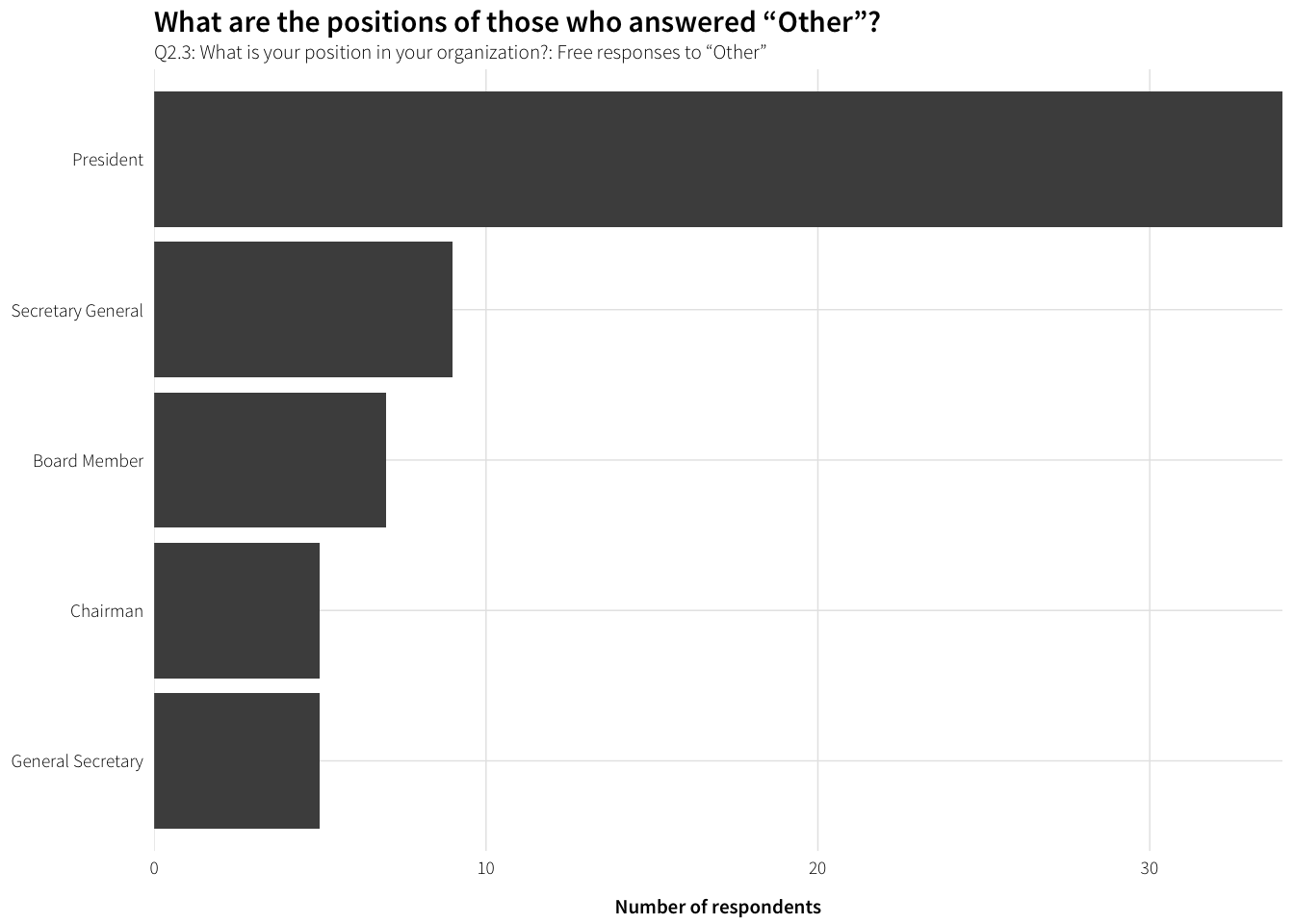### How are these NGOs distributed by HQ?

df.hq.countries <- survey.orgs.clean %>%
group_by(Q2.2_iso3) %>%
summarise(num.ngos = n()) %>%
ungroup() %>%
# Combine with list of all possible mappable countries
right_join(possible.countries, by=c("Q2.2_iso3"="id")) %>%
mutate(num.ceiling = ifelse(num.ngos >= 50, 50, num.ngos),
prop = num.ngos / sum(num.ngos, na.rm=TRUE),
country.name = countrycode(Q2.2_iso3, "iso3c", "country.name"),
presence = num.ngos >= 1) %>%
arrange(desc(num.ngos))

datatable(df.hq.countries)

sum(df.hq.countries$presence, na.rm=TRUE) ##  93 #### Regional distribution of HQ countries df.hq.regions <- df.hq.countries %>% filter(!is.na(num.ngos)) %>% mutate(region = countrycode(Q2.2_iso3, "iso3c", "continent"), region = ifelse(Q2.2_iso3 == "TWN", "Asia", region)) %>% group_by(region) %>% summarise(num = sum(num.ngos, na.rm=TRUE)) %>% ungroup() %>% mutate(prop = num / sum(num)) %>% arrange(desc(num)) %>% mutate(region = factor(region, levels=rev(region), ordered=TRUE)) df.hq.regions ## # A tibble: 5 × 3 ## region num prop ## <ord> <int> <dbl> ## 1 Europe 228 0.3613312 ## 2 Americas 195 0.3090333 ## 3 Africa 109 0.1727417 ## 4 Asia 85 0.1347068 ## 5 Oceania 14 0.0221870 plot.hq.regions <- ggplot(df.hq.regions, aes(x=num, y=region)) + geom_barh(stat="identity") + scale_x_continuous(expand=c(0, 0)) + labs(x="Number of respondents", y=NULL, title="Region of headquarters", subtitle="Q2.2: Where is your organization's headquarters? (summarized by region)") + theme_ath() plot.hq.regions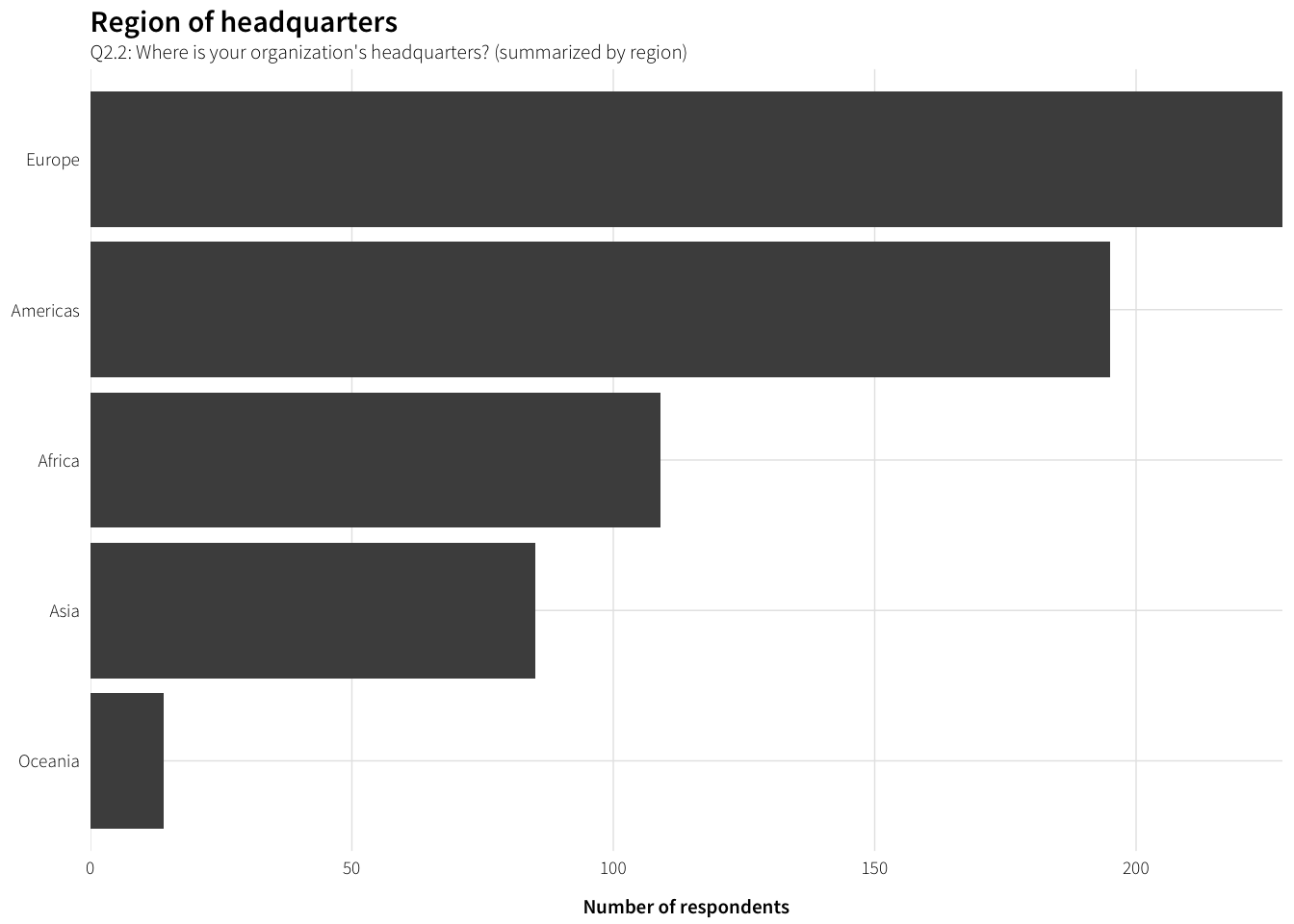#### Countries with at least one response plot.hq.map.presence <- ggplot(df.hq.countries, aes(fill=presence, map_id=Q2.2_iso3)) + geom_map(map=countries.ggmap, size=0.15, colour="black") + expand_limits(x=countries.ggmap$long, y=countries.ggmap$lat) + coord_equal() + scale_fill_manual(values=c("grey50", "#FFFFFF"), na.value="#FFFFFF", guide=FALSE) + labs(title="Countries with at least one response", subtitle="Q2.2: Where is your organization's headquarters?") + theme_ath_map() plot.hq.map.presence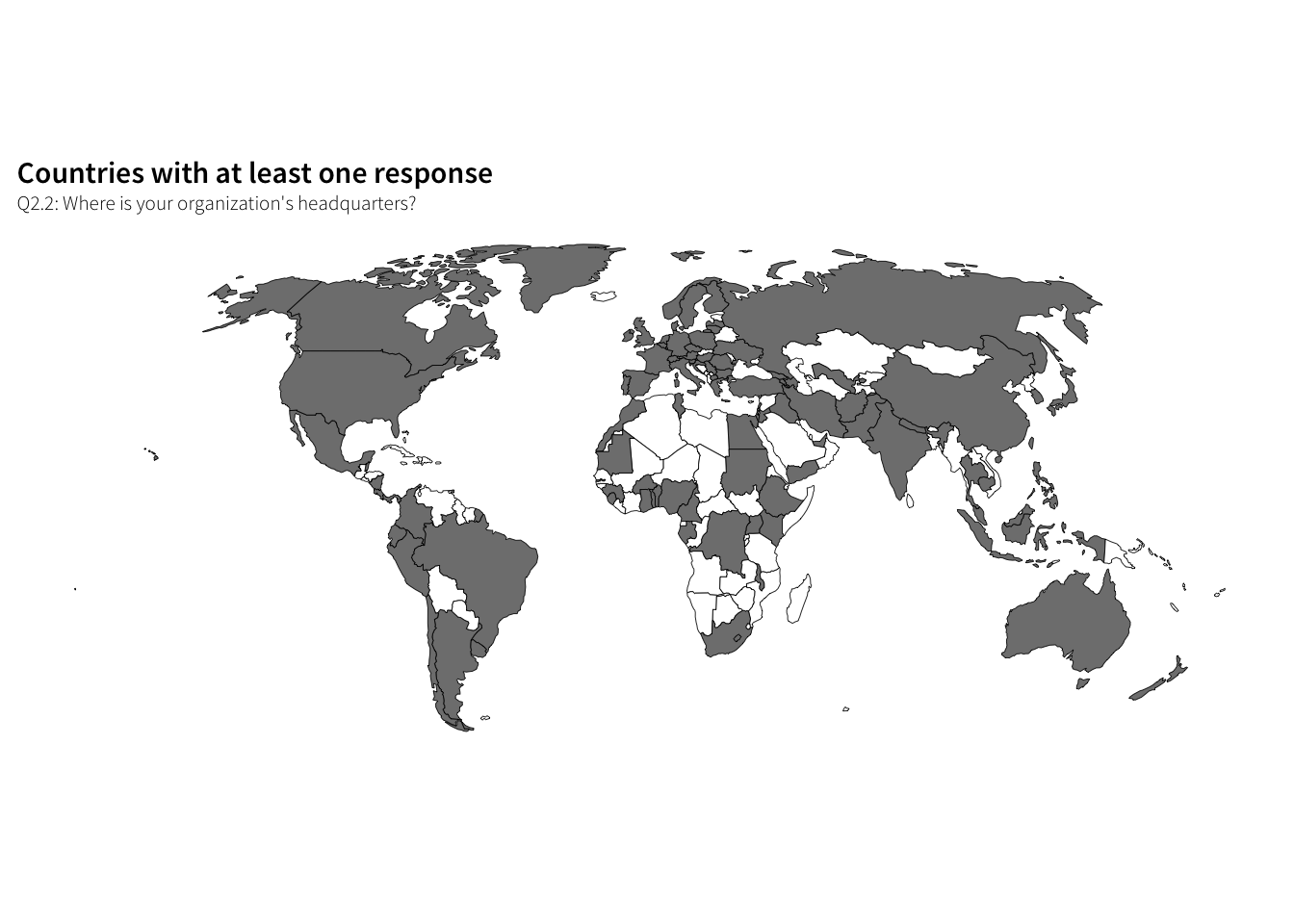#### Responses per country (50 NGO ceiling) plot.hq.map.scale <- ggplot(df.hq.countries, aes(fill=num.ceiling, map_id=Q2.2_iso3)) + geom_map(map=countries.ggmap, size=0.15, colour="black") + expand_limits(x=countries.ggmap$long, y=countries.ggmap$lat) + coord_equal() + scale_fill_gradient(low="grey95", high="grey20", breaks=seq(0, 50, 10), labels=c(paste(seq(0, 40, 10), " "), "50+"), na.value="#FFFFFF", name="NGOs based in country", guide=guide_colourbar(ticks=FALSE, barwidth=6)) + labs(title="Number of responding NGOs around the world", subtitle="Q2.2: Where is your organization's headquarters? (50 NGO ceiling)") + theme_ath_map() + theme(legend.position="bottom", legend.key.size=unit(0.65, "lines"), strip.background=element_rect(colour="#FFFFFF", fill="#FFFFFF")) plot.hq.map.scale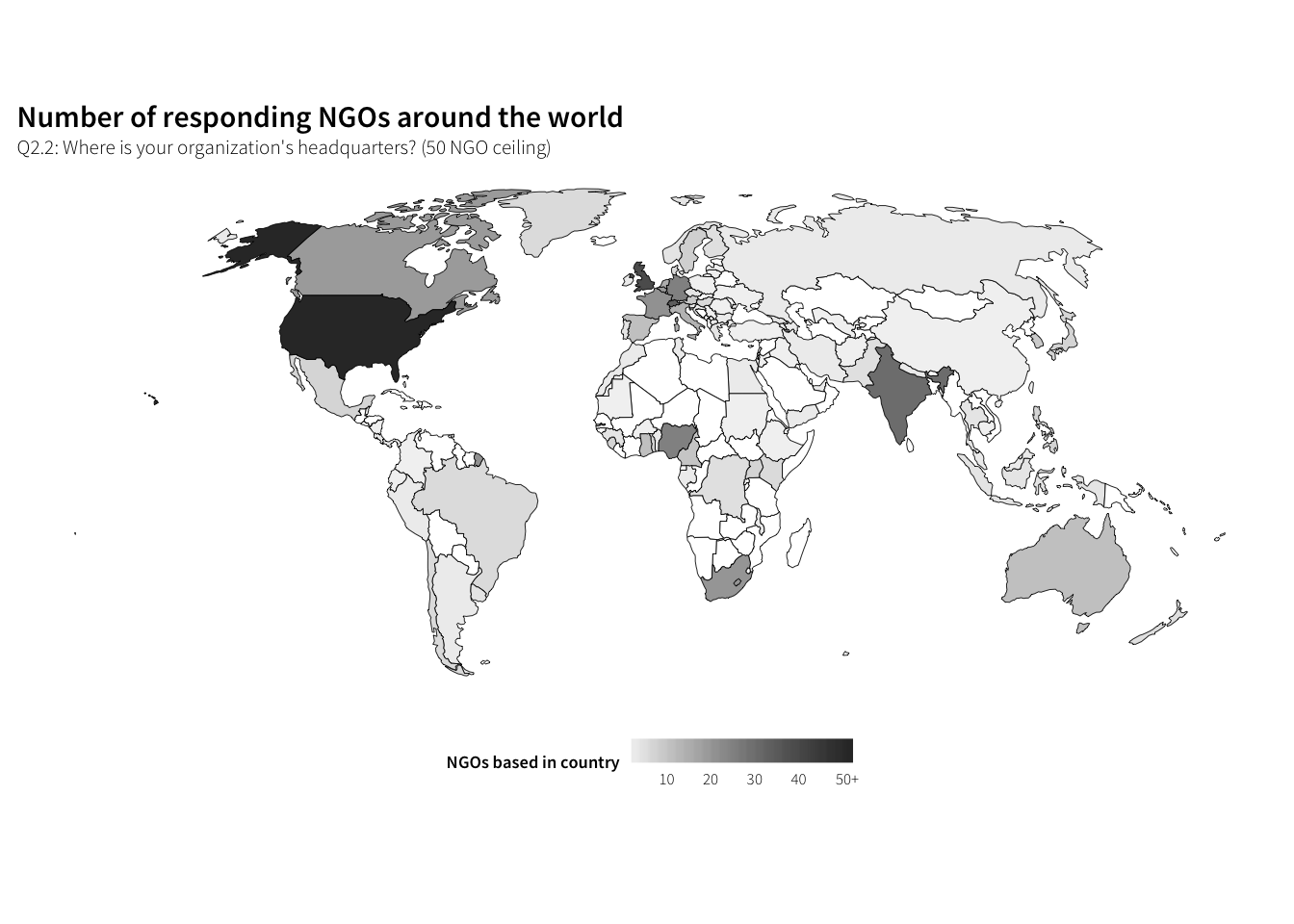### Where do these NGOs work? df.work.countries.all <- survey.orgs.clean %>% unnest(Q2.5_iso3) %>% group_by(Q2.5_iso3) %>% summarise(num = n()) %>% ungroup() %>% # Combine with list of all possible mappable countries right_join(possible.countries, by=c("Q2.5_iso3"="id")) %>% mutate(prop = num / sum(num, na.rm=TRUE), country.name = countrycode(Q2.5_iso3, "iso3c", "country.name"), presence = num >= 1) %>% arrange(desc(num)) datatable(df.work.countries.all) #### Regional distribution of countries where NGOs report working df.work.regions.all <- survey.orgs.clean %>% unnest(Q2.5_iso3) %>% mutate(region = countrycode(Q2.5_iso3, "iso3c", "continent"), region = ifelse(Q2.5_iso3 == "TWN", "Asia", region), region = ifelse(Q2.5_iso3 == "XKX", "Europe", region)) %>% group_by(region) %>% summarise(num = n()) %>% ungroup() %>% mutate(prop = num / sum(num)) %>% arrange(desc(num)) df.work.regions.all ## # A tibble: 5 × 3 ## region num prop ## <chr> <int> <dbl> ## 1 Europe 2988 0.2973430 ## 2 Africa 2625 0.2612200 ## 3 Asia 2346 0.2334561 ## 4 Americas 1735 0.1726540 ## 5 Oceania 355 0.0353269 Not plotting because the organization-county-region unit is a little wonky. #### Number of unique countries where work is reported sum(df.work.countries.all$presence, na.rm=TRUE)
##  169

That’s pretty much every country! There are 172 recognized countries in the map file, so mapping them out is kind of pointless.

#### Responses per country

plot.work.all.map.scale <- ggplot(df.work.countries.all,
aes(fill=num, map_id=Q2.5_iso3)) +
geom_map(map=countries.ggmap, size=0.15, colour="black") +
expand_limits(x=countries.ggmap$long, y=countries.ggmap$lat) +
coord_equal() +
# labels=c(paste(seq(0, 150, 50), "  "), "50+"),
na.value="#FFFFFF", name="NGOs reporting work in country",
guide=guide_colourbar(ticks=FALSE, barwidth=6)) +
labs(title="Countries where NGOs work",
subtitle="Q2.5: Besides 'home_country', where does your organization work?") +
theme_ath_map() +
theme(legend.position="bottom", legend.key.size=unit(0.65, "lines"),
strip.background=element_rect(colour="#FFFFFF", fill="#FFFFFF"))
plot.work.all.map.scale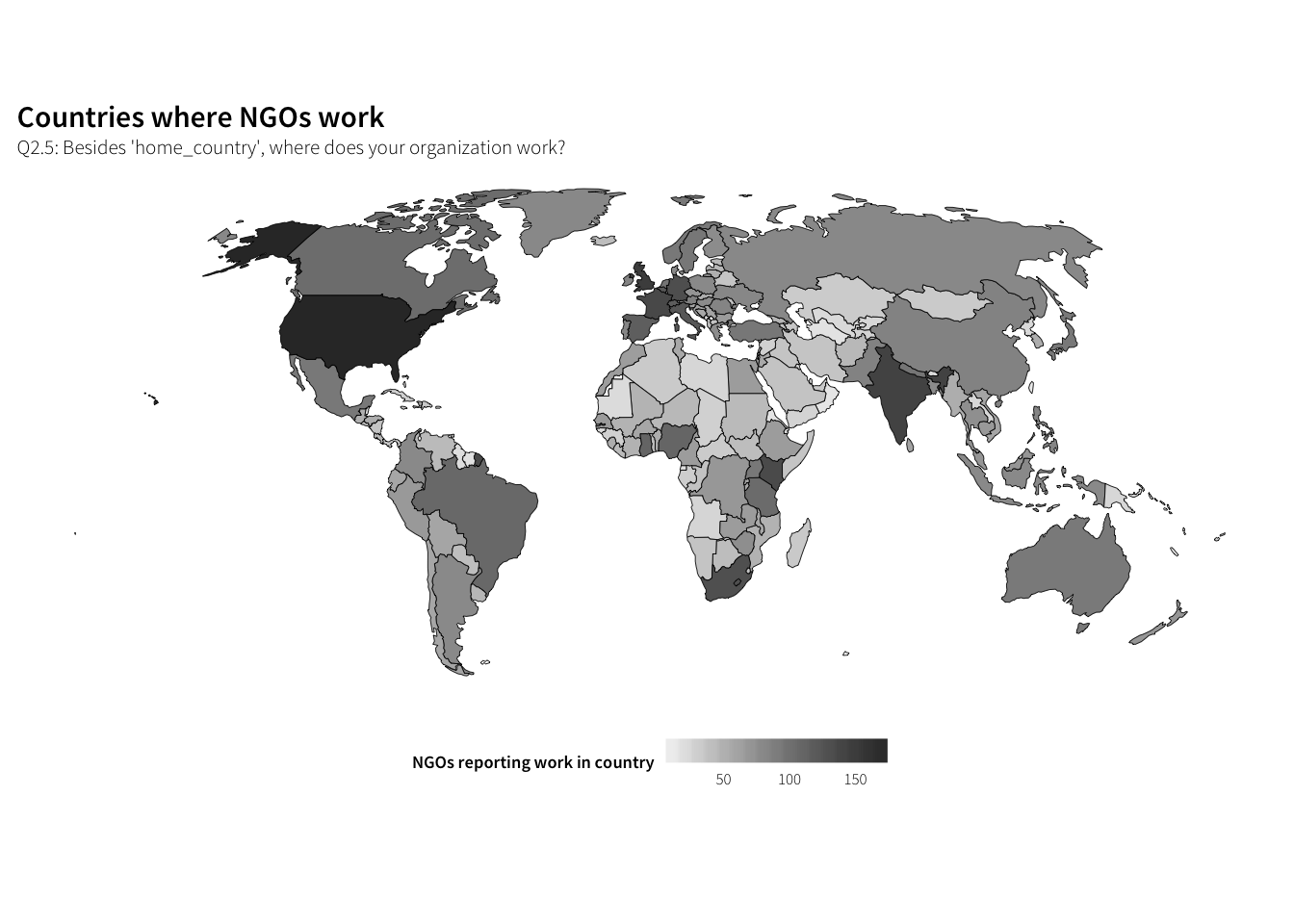df.work.countries.answered <- survey.countries.clean %>%
group_by(Q4.1_iso3) %>%
summarise(num = n()) %>%
ungroup() %>%
# Combine with list of all possible mappable countries
right_join(possible.countries, by=c("Q4.1_iso3"="id")) %>%
mutate(num.ceiling = ifelse(num >= 20, 20, num),
prop = num / sum(num, na.rm=TRUE),
country.name = countrycode(Q4.1_iso3, "iso3c", "country.name"),
presence = num >= 1) %>%
arrange(desc(num))

datatable(df.work.countries.answered)

df.work.regions.answered <- df.work.countries.answered %>%
filter(!is.na(num)) %>%
mutate(region = countrycode(Q4.1_iso3, "iso3c", "continent")) %>%
group_by(region) %>%
summarise(num = sum(num)) %>% ungroup() %>%
mutate(prop = num / sum(num)) %>%
arrange(desc(num)) %>%
mutate(region = factor(region, levels=rev(region), ordered=TRUE))
df.work.regions.answered
## # A tibble: 5 × 3
##     region   num      prop
##      <ord> <int>     <dbl>
## 1   Africa   200 0.3105590
## 2     Asia   158 0.2453416
## 3   Europe   151 0.2344720
## 4 Americas   115 0.1785714
## 5  Oceania    20 0.0310559
plot.work.regions <- ggplot(df.work.regions.answered, aes(x=num, y=region)) +
geom_barh(stat="identity") +
scale_x_continuous(expand=c(0, 0)) +
labs(x="Number of responses", y=NULL,
subtitle="One of countries selected in Q2.5: Besides 'home_country', where does your organization work?") +
theme_ath()
plot.work.regions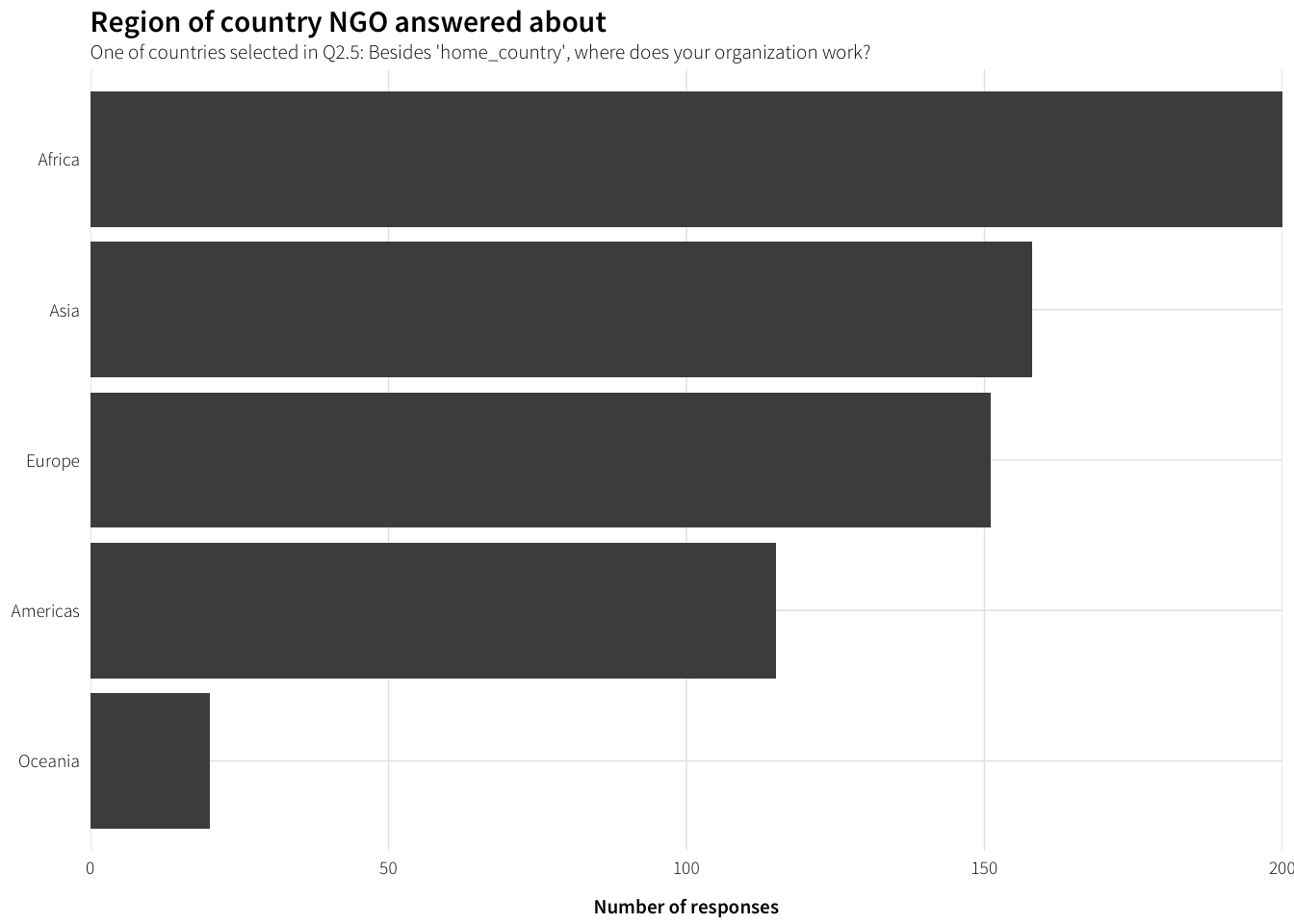sum(df.work.countries.answered$presence, na.rm=TRUE) ##  139 #### Countries with at least one response plot.work.map.presence <- ggplot(df.work.countries.answered, aes(fill=presence, map_id=Q4.1_iso3)) + geom_map(map=countries.ggmap, size=0.15, colour="black") + expand_limits(x=countries.ggmap$long, y=countries.ggmap$lat) + coord_equal() + scale_fill_manual(values=c("grey50", "#FFFFFF"), na.value="#FFFFFF", guide=FALSE) + labs(title="Countries about which at least one NGO answered questions", subtitle="Q4.1: Please select a country you would like to discuss\n(One of countries selected in Q2.5)") + theme_ath_map() plot.work.map.presence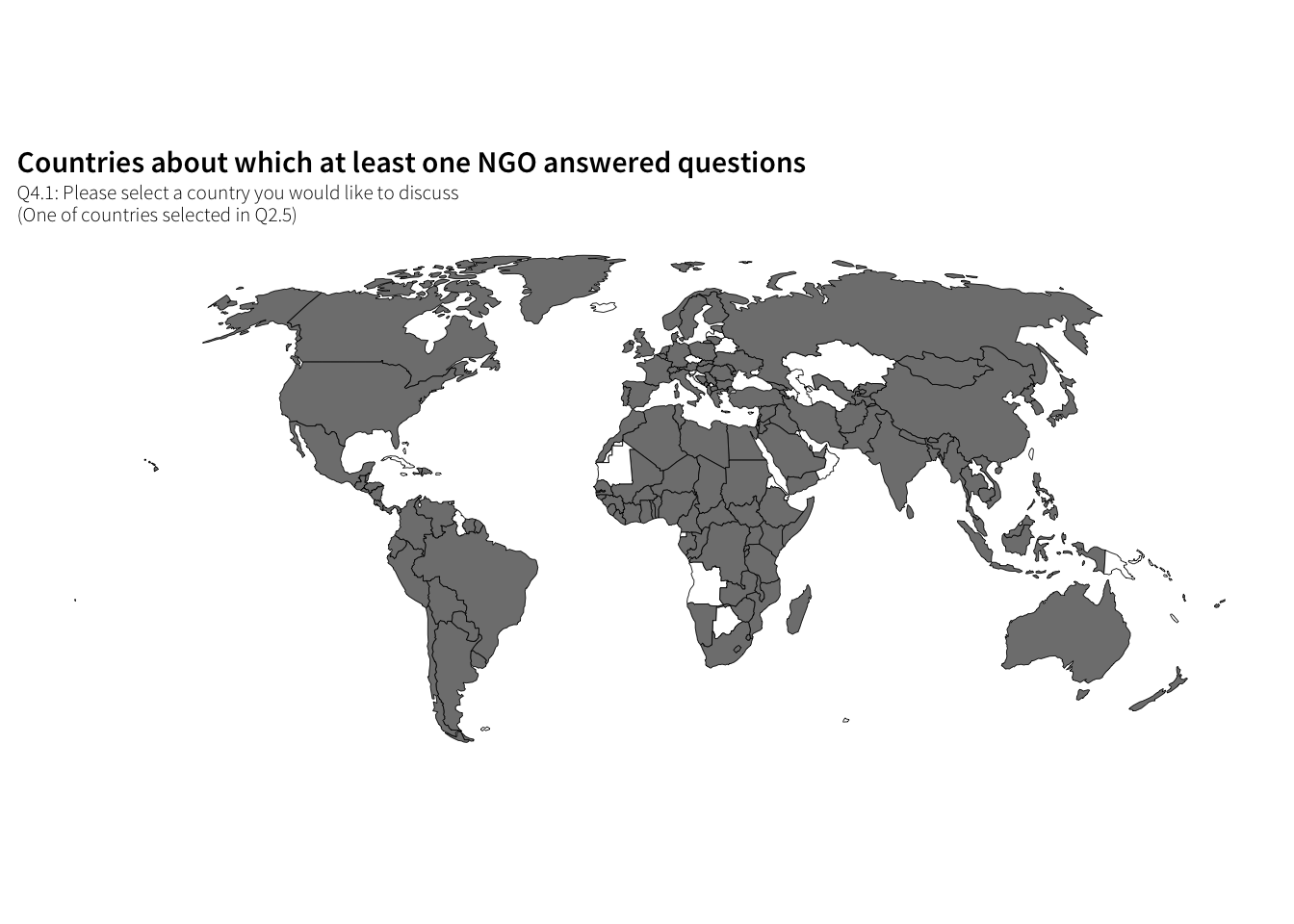# #### Responses per country (20 NGO ceiling) plot.work.map.scale <- ggplot(df.work.countries.answered, aes(fill=num.ceiling, map_id=Q4.1_iso3)) + geom_map(map=countries.ggmap, size=0.15, colour="black") + expand_limits(x=countries.ggmap$long, y=countries.ggmap$lat) + coord_equal() + scale_fill_gradient(low="grey95", high="grey20", #breaks=seq(0, 50, 10), na.value="#FFFFFF", name="NGOs answered about country", guide=guide_colourbar(ticks=FALSE, barwidth=6)) + labs(title="Countries about which NGOs answered questions", subtitle="Q4.1: Please select a country you would like to discuss\n(One of countries selected in Q2.5)") + theme_ath_map() + theme(legend.position="bottom", legend.key.size=unit(0.65, "lines"), strip.background=element_rect(colour="#FFFFFF", fill="#FFFFFF")) plot.work.map.scale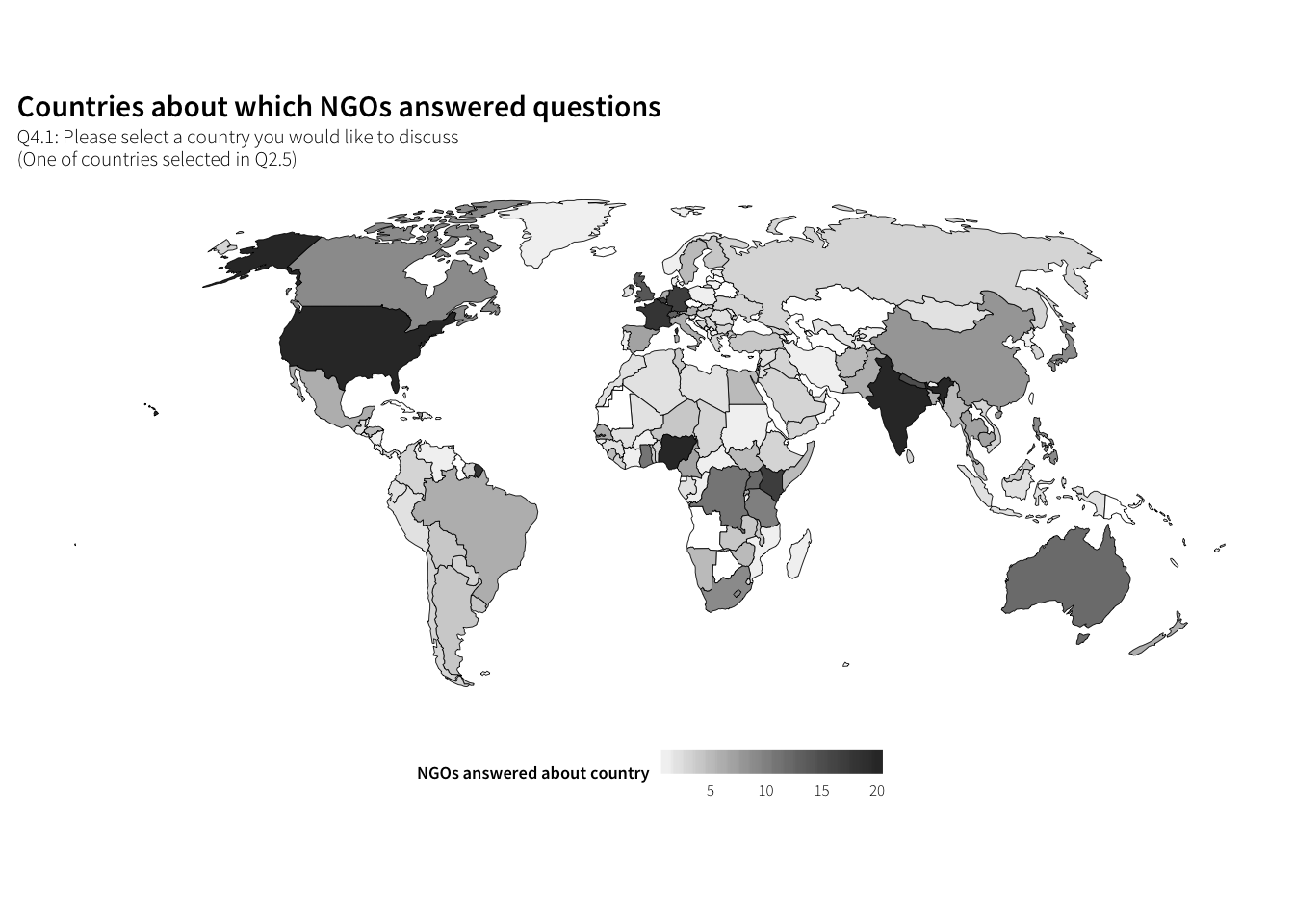## What do these NGOs do? ### Which issues do these NGOs work on? df.issues <- survey.orgs.clean %>% unnest(Q3.1_value) %>% group_by(Q3.1_value) %>% summarise(num = n()) %>% arrange(desc(num)) %>% filter(!is.na(Q3.1_value)) %>% mutate(issue = factor(Q3.1_value, levels=rev(Q3.1_value), ordered=TRUE)) plot.issues <- ggplot(df.issues, aes(x=num, y=issue)) + geom_barh(stat="identity") + scale_x_continuous(expand=c(0, 0)) + labs(x="Times selected", y=NULL, title="Which issues do NGOs work on?", subtitle="Q3.1: Which issues does your organization focus on? (multiple answers allowed)") + theme_ath() plot.issues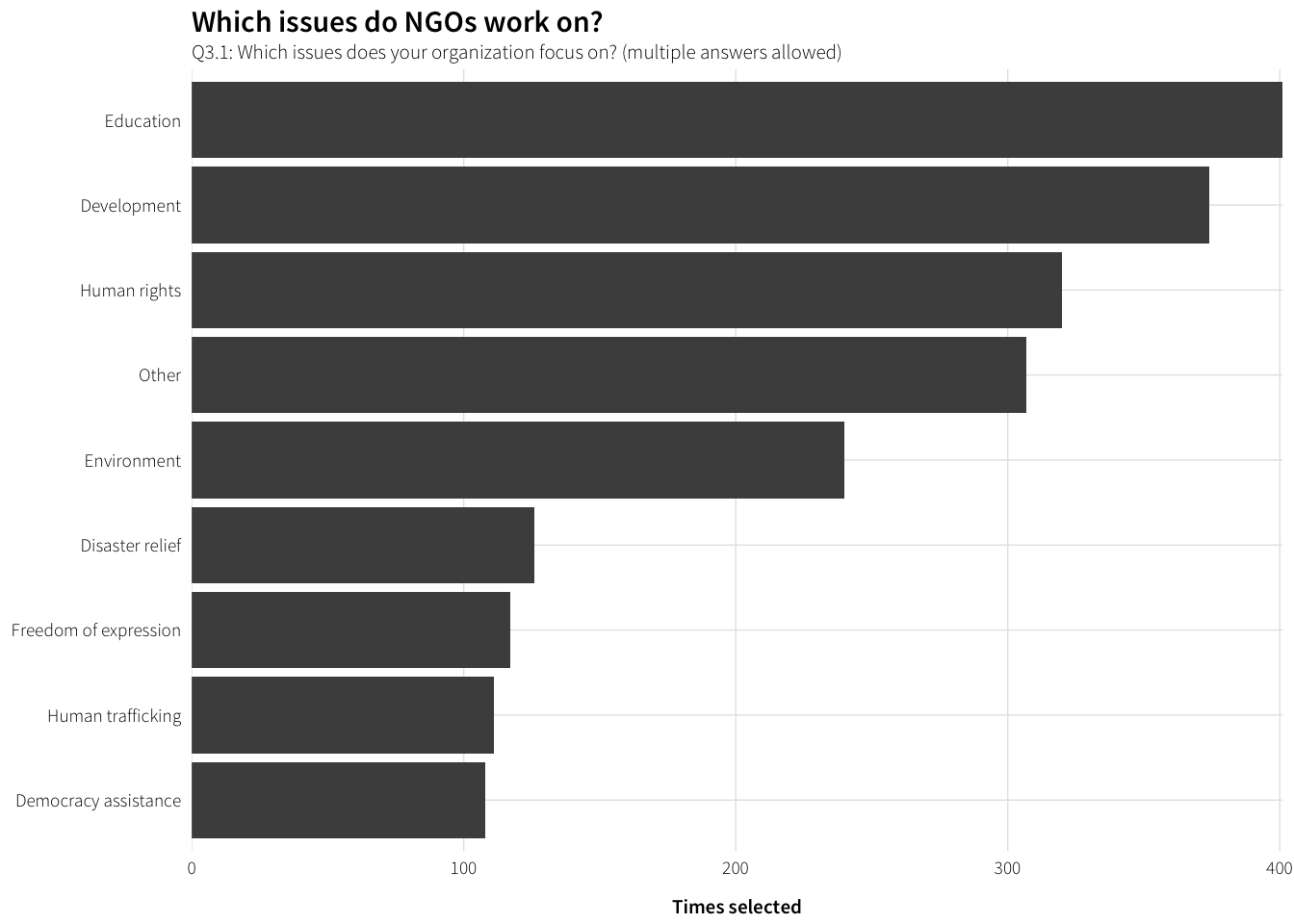### Which issues do NGOs work on the most? df.issues.most <- survey.orgs.clean %>% group_by(Q3.2) %>% summarise(num = n()) %>% arrange(desc(num)) %>% filter(!is.na(Q3.2)) %>% mutate(issue = factor(Q3.2, levels=rev(Q3.2), ordered=TRUE)) plot.issues.most <- ggplot(df.issues.most, aes(x=num, y=issue)) + geom_barh(stat="identity") + scale_x_continuous(expand=c(0, 0)) + labs(x="Times selected", y=NULL, title="Which issues do NGOs work on?", subtitle="Q3.2: Which issues does your organization focus on the most?") + theme_ath() plot.issues.most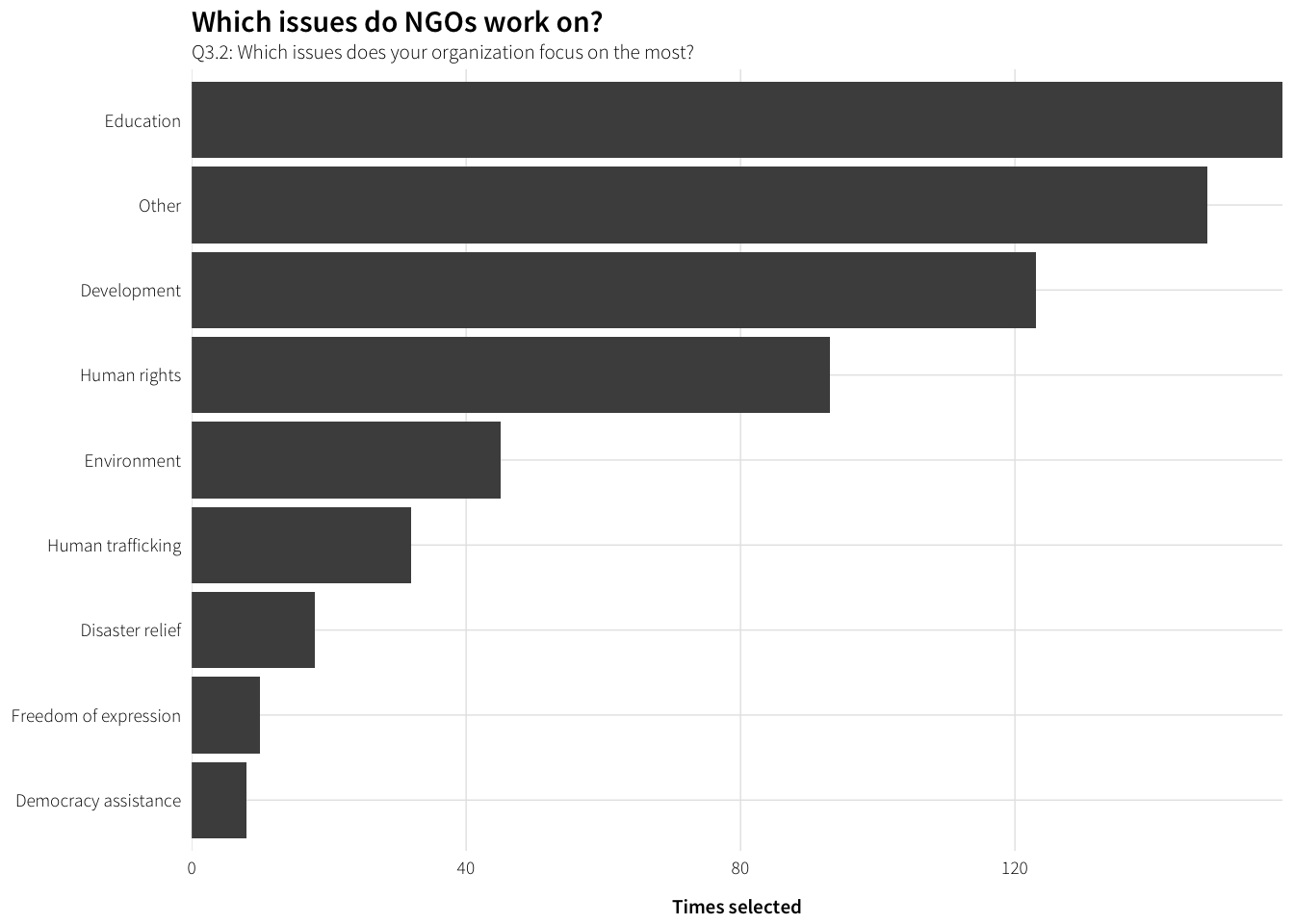There are lots of others, both for all issues and most important issue. What are they? df.other.issues <- survey.orgs.clean %>% filter(!is.na(Q3.1_other_TEXT)) %>% mutate(other.issue = str_to_title(Q3.1_other_TEXT)) %>% group_by(other.issue) %>% summarise(num = n()) %>% arrange(desc(num)) datatable(df.other.issues) There are 300+ other issues here. That’s a lot. Natural language processing can help make sense of the mess. # Build clean corpus for NLP issue.corpus.df <- survey.orgs.clean %>% filter(!is.na(Q3.1_other_TEXT)) %>% mutate(other.issue = str_to_lower(Q3.1_other_TEXT)) %>% select(other.issue) issue.corpus <- issue.corpus.df %>% DataframeSource() %>% Corpus() %>% tm_map(removePunctuation) %>% tm_map(removeNumbers) %>% tm_map(removeWords, stopwords("english")) %>% tm_map(stemDocument, language="english") %>% tm_map(stripWhitespace) tdm.issues <- TermDocumentMatrix(issue.corpus) # This works, but doesn't provide frequencies, so dplyr it is # findFreqTerms(tdm.issues, 5) term.frequency <- as.data.frame(as.matrix(tdm.issues)) %>% mutate(term = rownames(.)) %>% gather(document, freq, -term) %>% filter(freq > 0) %>% group_by(term) %>% summarise(num = n()) %>% ungroup() frequent.issues <- term.frequency %>% filter(num >= 5) %>% arrange(desc(num)) frequent.issues ## # A tibble: 39 × 2 ## term num ## <chr> <int> ## 1 health 45 ## 2 develop 17 ## 3 right 17 ## 4 women 15 ## 5 cultur 11 ## 6 youth 11 ## 7 children 10 ## 8 educ 10 ## 9 research 10 ## 10 intern 9 ## # ... with 29 more rows Lots of NGOs deal with rights, health, and developent. Do they qualify those broader issues? findAssocs(tdm.issues, "right", 0.1) ##$right
##        csr instrument     mechan strengthen      human      minor
##       0.42       0.42       0.42       0.42       0.35       0.28
##   children     intern      women      legal     achiev       arab
##       0.26       0.25       0.23       0.22       0.20       0.20
##     aspect  audiovisu       busi      child    citizen   creation
##       0.20       0.20       0.20       0.20       0.20       0.20
##     hivaid intellectu        kid   properti     regard    societi
##       0.20       0.20       0.20       0.20       0.20       0.20
##     talent       type     victim    migrant     justic       hous
##       0.20       0.20       0.20       0.19       0.16       0.13
##     improv     integr     labour       life      peopl    special
##       0.13       0.13       0.13       0.13       0.13       0.13
##    protect    exchang      focus     prison
##       0.11       0.10       0.10       0.10
findAssocs(tdm.issues, "health", 0.1)
## $health ## primari hiv reconcili reproduct ## 0.23 0.19 0.19 0.19 ## sexual access cititenship diabet ## 0.19 0.13 0.13 0.13 ## divers enterpri environment extracurricular ## 0.13 0.13 0.13 0.13 ## finncial hivaid iaid incluion ## 0.13 0.13 0.13 0.13 ## inclus laser less lifestyl ## 0.13 0.13 0.13 0.13 ## literaci much pauvreti privileg ## 0.13 0.13 0.13 0.13 ## reductionn safeti promot care ## 0.13 0.13 0.11 0.10 findAssocs(tdm.issues, "develop", 0.1) ##$develop
##       address        better         chain      communic        effici
##          0.24          0.24          0.24          0.24          0.24
##      enterpri     entertain      finncial       harmoni      incluion
##          0.24          0.24          0.24          0.24          0.24
##          less         lobbi           non           one     peacekeep
##          0.24          0.24          0.24          0.24          0.24
##         polit        privat      privileg         renew       researh
##          0.24          0.24          0.24          0.24          0.24
##        sector        volunt         world        econom        energi
##          0.24          0.24          0.24          0.19          0.18
##         capac     communiti        diseas           etc     healthcar
##          0.16          0.16          0.16          0.16          0.16
##           ict infrastructur         skill         trade          valu
##          0.16          0.16          0.16          0.16          0.16
##    peacebuild        intern       control         focus    interfaith
##          0.15          0.13          0.12          0.12          0.12
##       primari       program          wash         youth
##          0.12          0.12          0.12          0.11

Imputing latent themes from these other topics algorithmically is tricky though. K-means, PAM, latent semantic analysis (LSA), and latent dirichlet allocation (LDA) all choke, since the corpus isn’t that big and the “documents” are super short (often just one word long). I attempt each of these in /Analysis/ingo_survey/sandbox.R, but none of them work well, even for coarse sorting.

So, hand-coding it is.

Here are the results post-hand-coding:

df.issues.clean <- survey.orgs.clean %>%
unnest(Q3.1.clean_value) %>%
group_by(Q3.1.clean_value) %>%
summarise(num = n()) %>%
arrange(desc(num)) %>%
filter(!is.na(Q3.1.clean_value)) %>%
mutate(issue = factor(Q3.1.clean_value, levels=rev(Q3.1.clean_value), ordered=TRUE))

plot.issues.clean <- ggplot(df.issues.clean, aes(x=num, y=issue)) +
geom_barh(stat="identity") +
scale_x_continuous(expand=c(0, 0)) +
labs(x="Times selected", y=NULL,
title="Which issues do NGOs work on?",
subtitle="Q3.1: Which issues does your organization focus on? (multiple answers allowed)") +
theme_ath()

plot.issues.clean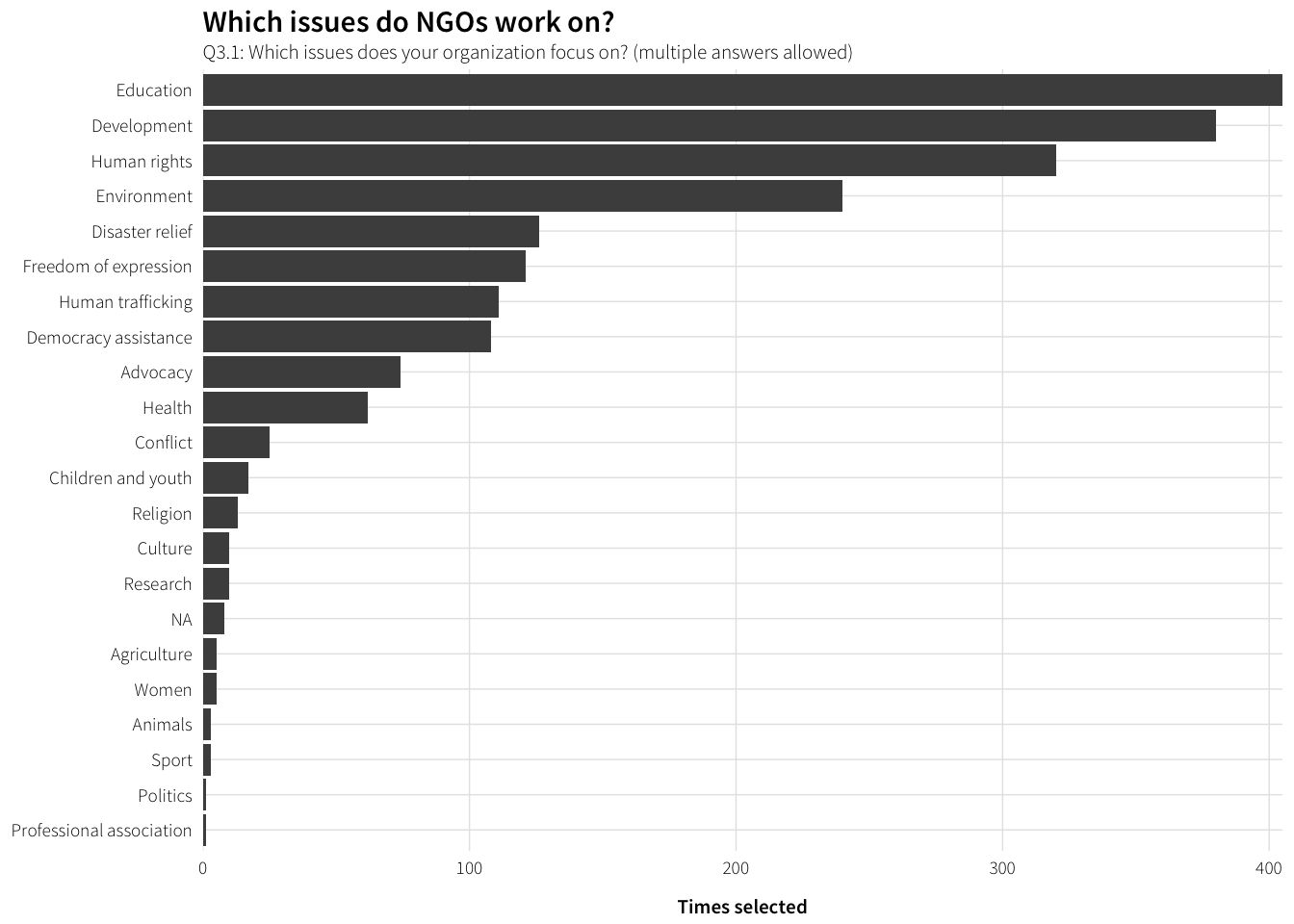df.issues.most.clean <- survey.orgs.clean %>%
group_by(Q3.2.clean, potential.contentiousness) %>%
summarise(num = n()) %>%
filter(!is.na(Q3.2.clean)) %>%
ungroup() %>%
arrange(desc(num)) %>%
mutate(issue = factor(Q3.2.clean, levels=rev(unique(Q3.2.clean)), ordered=TRUE))

plot.issues.most.clean <- ggplot(df.issues.most.clean,
aes(x=num, y=issue,
fill=potential.contentiousness)) +
geom_barh(stat="identity") +
scale_x_continuous(expand=c(0, 0)) +
scale_fill_manual(values=c("grey80", "grey40"),
name="Potential contentiousness") +
labs(x="Times selected", y=NULL,
title="Which issues do NGOs work on?",
subtitle="Q3.2: Which issues does your organization focus on the most?") +
theme_ath()

plot.issues.most.clean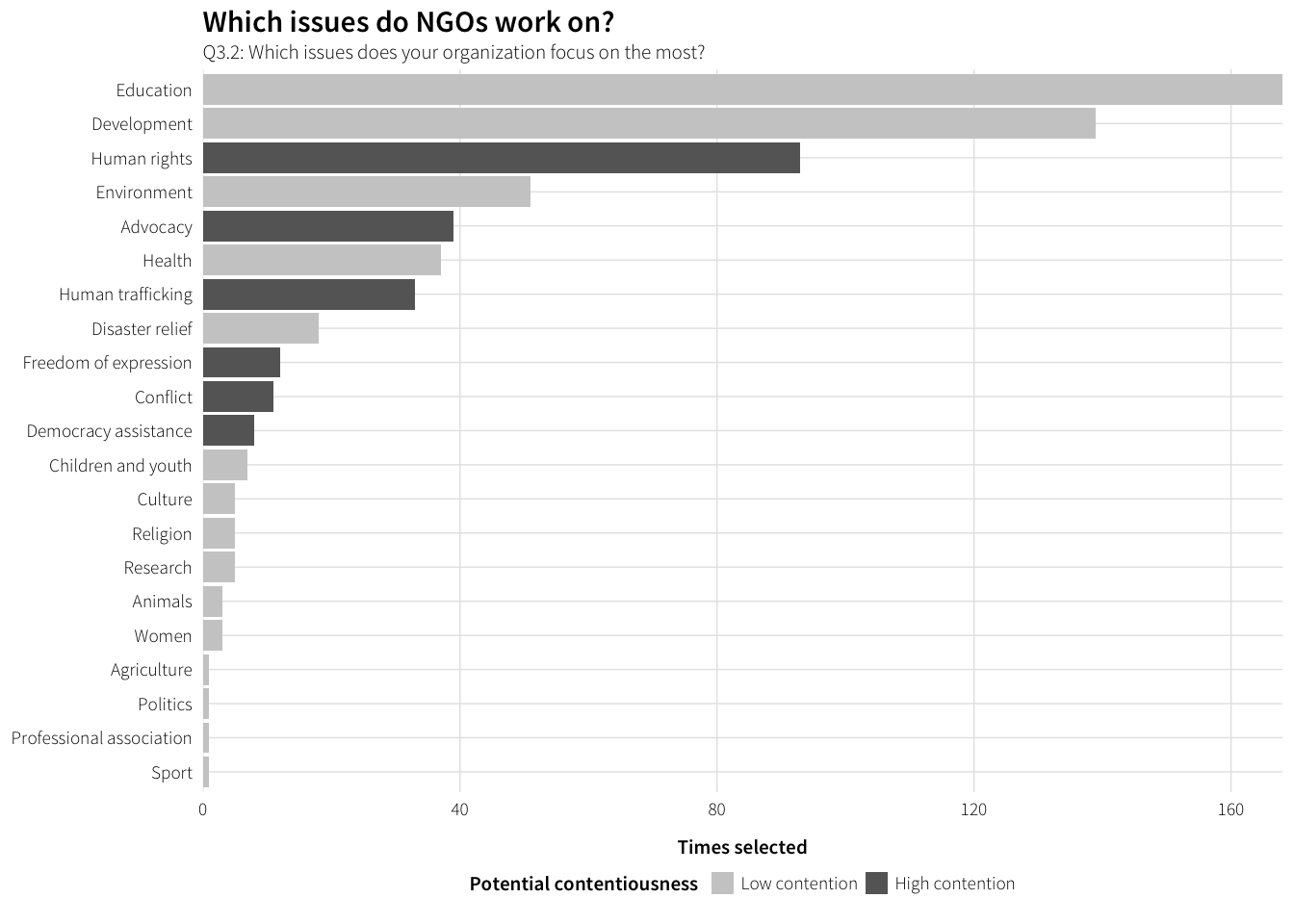### What kinds of activities do these NGOs engage in?

labels.activities <- data_frame(levels=c("aid", "education", "mobilize",
labels=c("Providing direct aid and services",
"Engaging in research and public education",
"Mobilizing people",
"Monitoring and assessing the effects of policies"))

df.activities <- survey.orgs.clean %>%
select(dplyr::contains("Q3.3"), -dplyr::contains("TEXT")) %>%
gather(question, response) %>%
mutate(question = str_replace(question, "Q3\\.3_", ""),
question = factor(question, levels=labels.activities$levels, labels=labels.activities$labels, ordered=TRUE)) %>%
filter(!(response %in% c("Don't know", "Not applicable"))) %>%
group_by(question, response) %>%
summarise(num = n()) %>%
ungroup() %>%
mutate(response = factor(response, levels=levels(survey.orgs.clean$Q3.3_aid), ordered=TRUE)) plot.activities <- ggplot(df.activities, aes(y=num, x=response)) + geom_bar(stat="identity") + labs(y="Number of responses", x=NULL, title="What kinds of activities are NGOs engaged in?", subtitle="Q3.3: Please indicate how often your organization engages in each of these types of activities") + facet_wrap(~ question, ncol=1) + theme_ath() plot.activities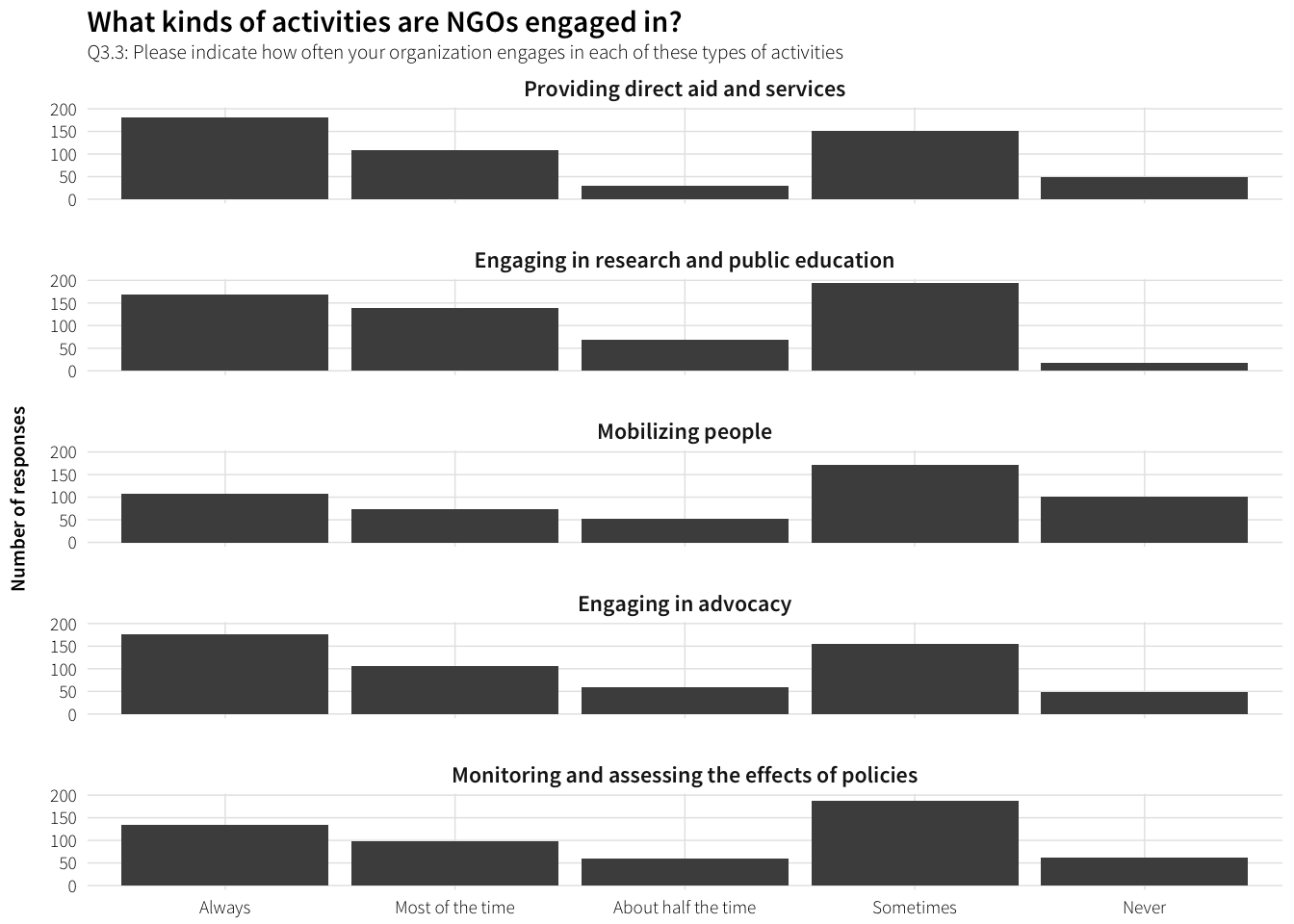### Staffing details #### Full time employees df.employees <- survey.orgs.clean %>% select(Q3.4.num) %>% filter(!is.na(Q3.4.num)) plot.employees <- ggplot(df.employees, aes(x=Q3.4.num)) + geom_histogram(binwidth=0.5) + labs(x="Number of employees (natural log)", y="Frequency", title="How many employees do NGOs use?", subtitle="Q3.4: Approximately how many full-time employees does your organization have?") + scale_x_continuous(trans="log1p", breaks=c(0, 10^(0:5)), labels=comma) + theme_ath() plot.employees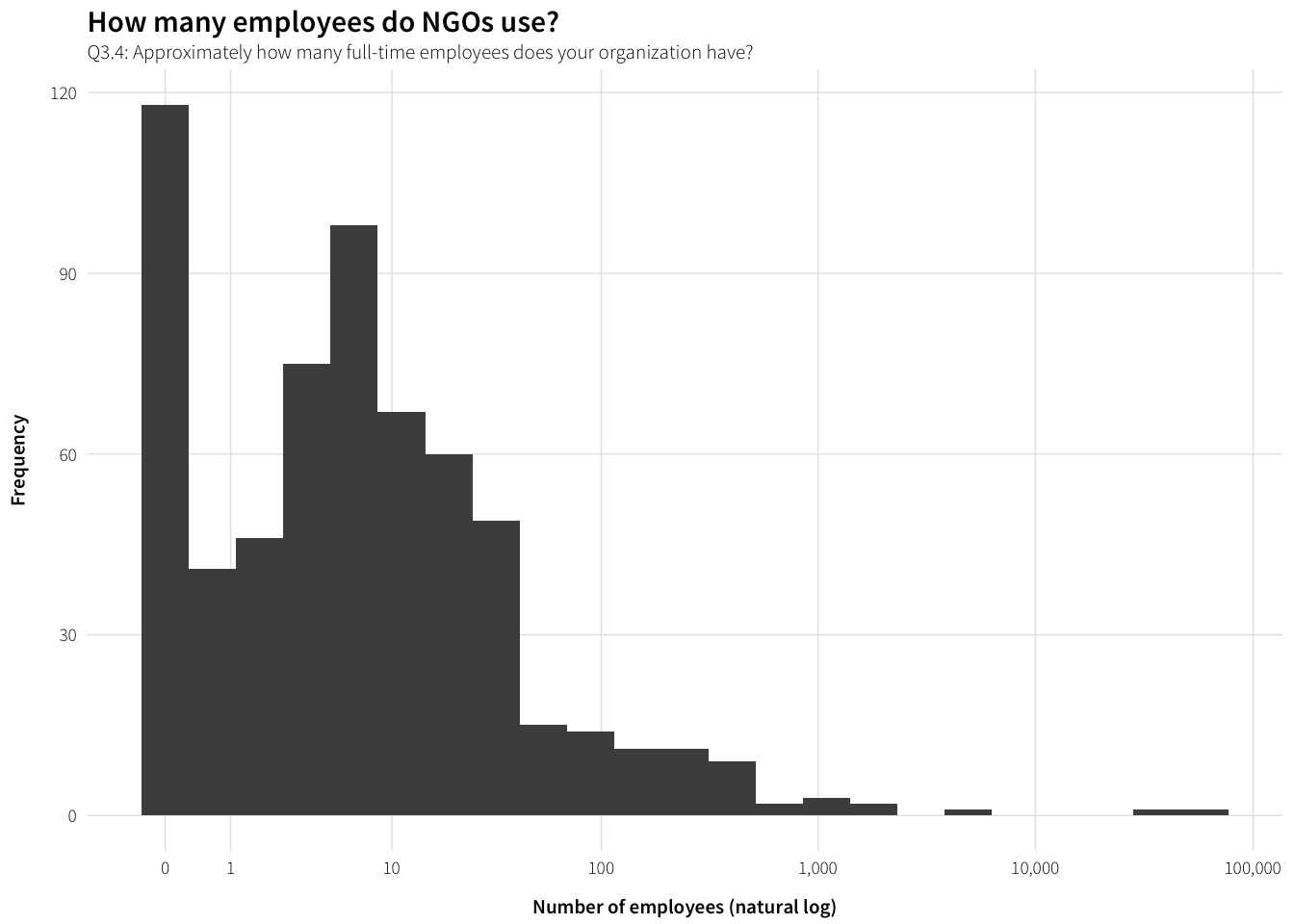#### Volunteers df.volunteers <- survey.orgs.clean %>% select(Q3.5.num) %>% filter(!is.na(Q3.5.num)) plot.volunteers <- ggplot(df.volunteers, aes(x=Q3.5.num)) + geom_histogram(binwidth=0.5) + labs(x="Number of volunteers (natural log)", y="Frequency", title="How many volunteers do NGOs use?", subtitle="Q3.5: Approximately how many volunteers does your organization have?") + scale_x_continuous(trans="log1p", breaks=c(0, 10^(0:6), 5000000), labels=comma) + theme_ath() plot.volunteers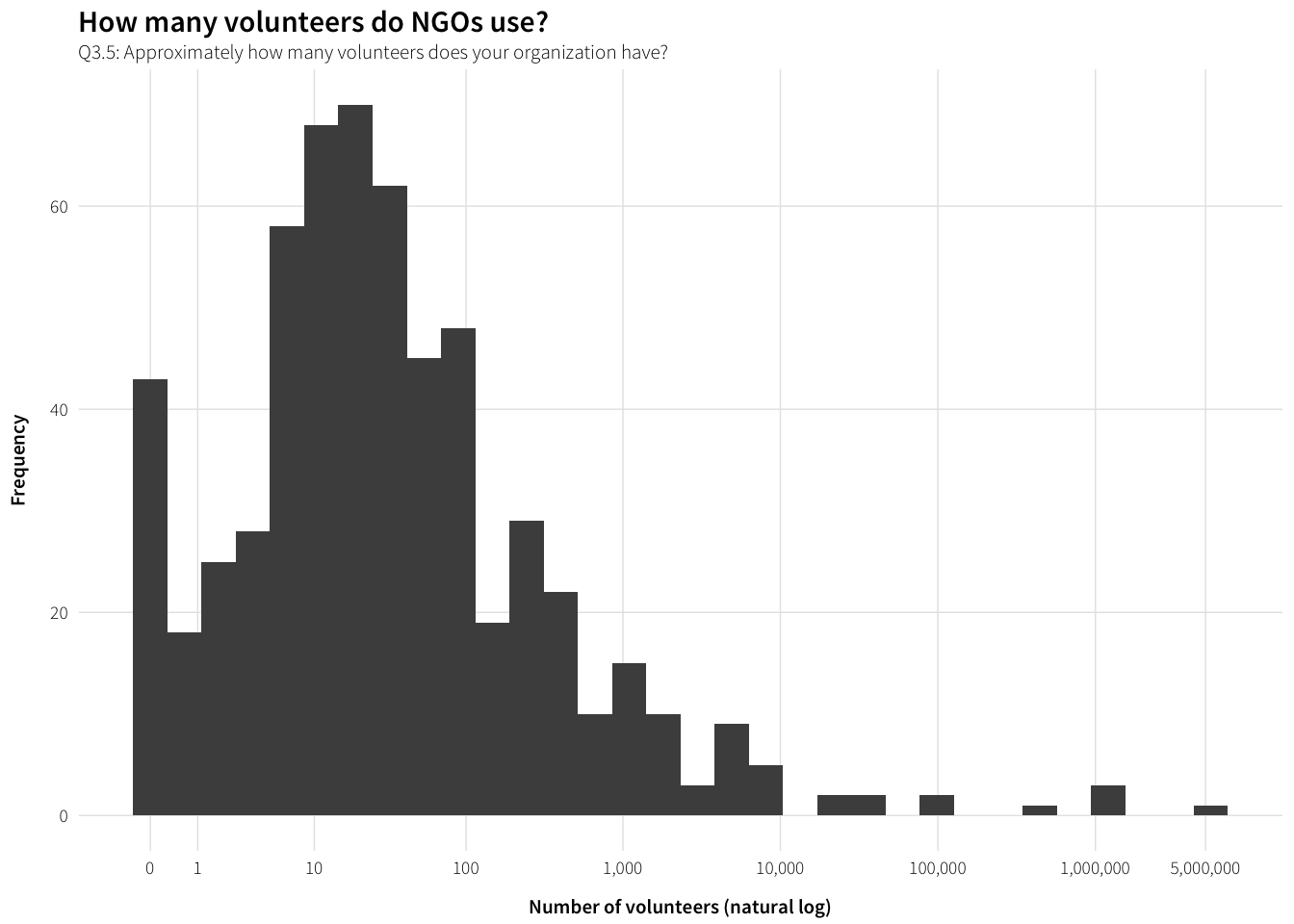### Collaboration df.collaboration <- survey.orgs.clean %>% unnest(Q3.6_value) %>% group_by(Q3.6_value) %>% summarise(num = n()) %>% arrange(desc(num)) %>% filter(!is.na(Q3.6_value)) %>% mutate(partner = factor(Q3.6_value, levels=rev(Q3.6_value), ordered=TRUE)) # Add line break to label levels(df.collaboration$partner)[levels(df.collaboration$partner) == "We do not collaborate with other organizations or institutions"] <- "We do not collaborate with other\norganizations or institutions" plot.collaboration <- ggplot(df.collaboration, aes(x=num, y=partner)) + geom_barh(stat="identity") + scale_x_continuous(expand=c(0, 0)) + labs(x="Times selected", y=NULL, title="Do NGOs collaborate with other organizations?", subtitle="Q3.6: Does your organization collaborate with any of these organizations\nor institutions? (multiple answers allowed)") + theme_ath() plot.collaboration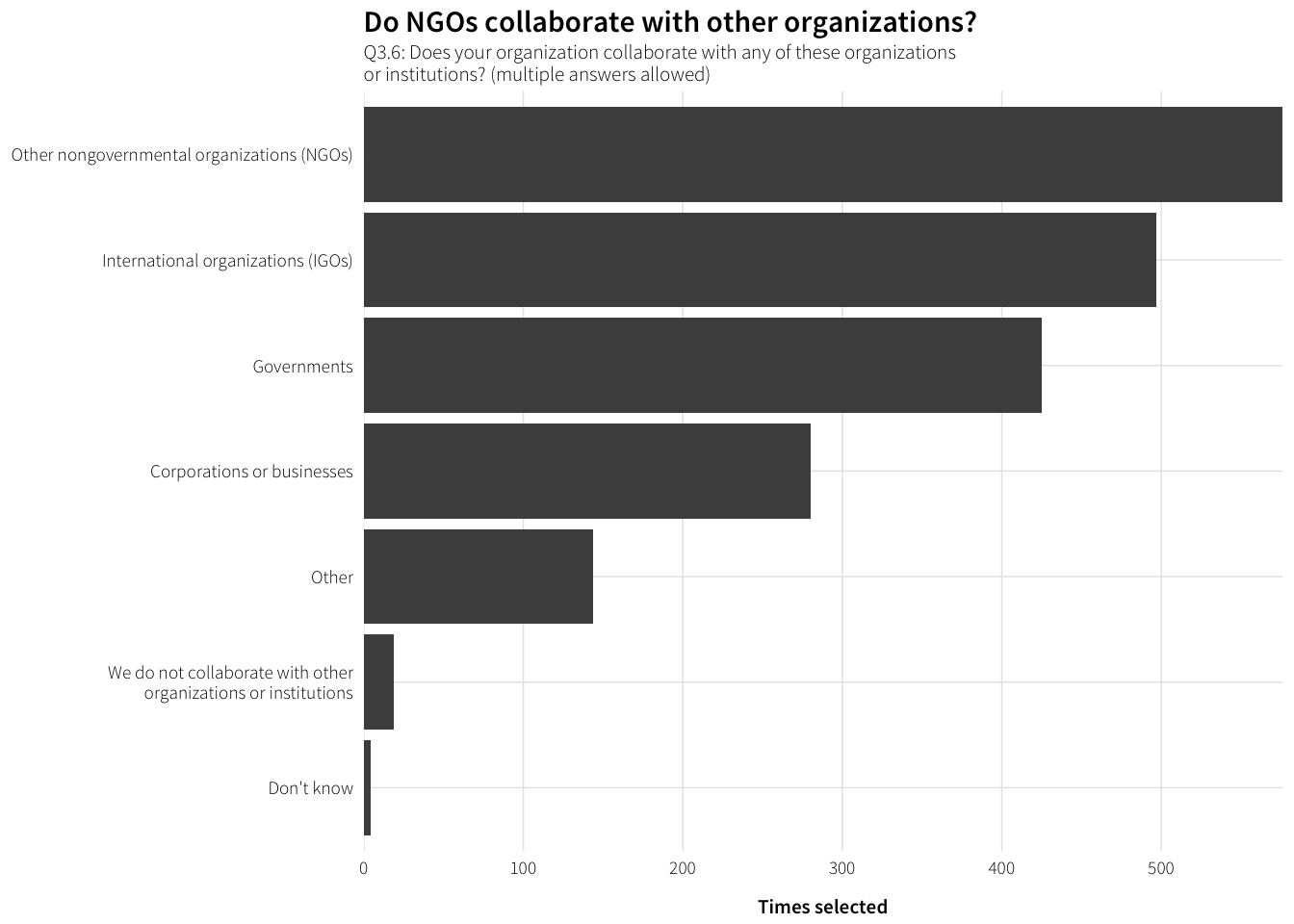What are the other organizations? df.collaboration.other <- survey.orgs.clean %>% filter(!is.na(Q3.6_other_TEXT)) %>% mutate(collaboration.other = str_to_title(Q3.6_other_TEXT)) %>% group_by(collaboration.other) %>% summarise(num = n()) %>% arrange(desc(num)) datatable(df.collaboration.other) Seems to mostly be universities, research centers, foundations, and religious groups. #### Specific organizations and institutions Q3.7: Please list a few of the organizations or institutions you partner with most often: df.collaboration.partners <- survey.orgs.clean %>% filter(!is.na(Q3.7)) %>% select(Q3.7) %>% arrange(Q3.7) datatable(df.collaboration.partners) ### Funding labels.funding <- data_frame(levels=c("individual", "corporate", "foundation", "home_govt", "host_govt", "other"), labels=c("Individual donations", "Corporate donations", "Foundation donations", "Grants from home country", "Grants from host country", "Other")) df.funding <- survey.orgs.clean %>% select(dplyr::contains("Q3.8"), -dplyr::contains("TEXT")) %>% gather(question, response) %>% mutate(question = str_replace(question, "Q3\\.8_", ""), question = factor(question, levels=labels.funding$levels,
labels=labels.funding$labels, ordered=TRUE)) %>% filter(!(response %in% c("Don't know", "Not applicable"))) %>% group_by(question, response) %>% summarise(num = n()) %>% ungroup() %>% mutate(response = factor(response, levels=levels(survey.orgs.clean$Q3.8_individual),
ordered=TRUE))

plot.funding <- ggplot(df.funding, aes(y=num, x=response)) +
geom_bar(stat="identity") +
labs(y="Number of responses", x=NULL,
title="Where does NGO funding come from?",
subtitle="Q3.8: How much of your organization’s funding comes from each of these sources?") +
facet_wrap(~ question, ncol=1) +
theme_ath()

plot.funding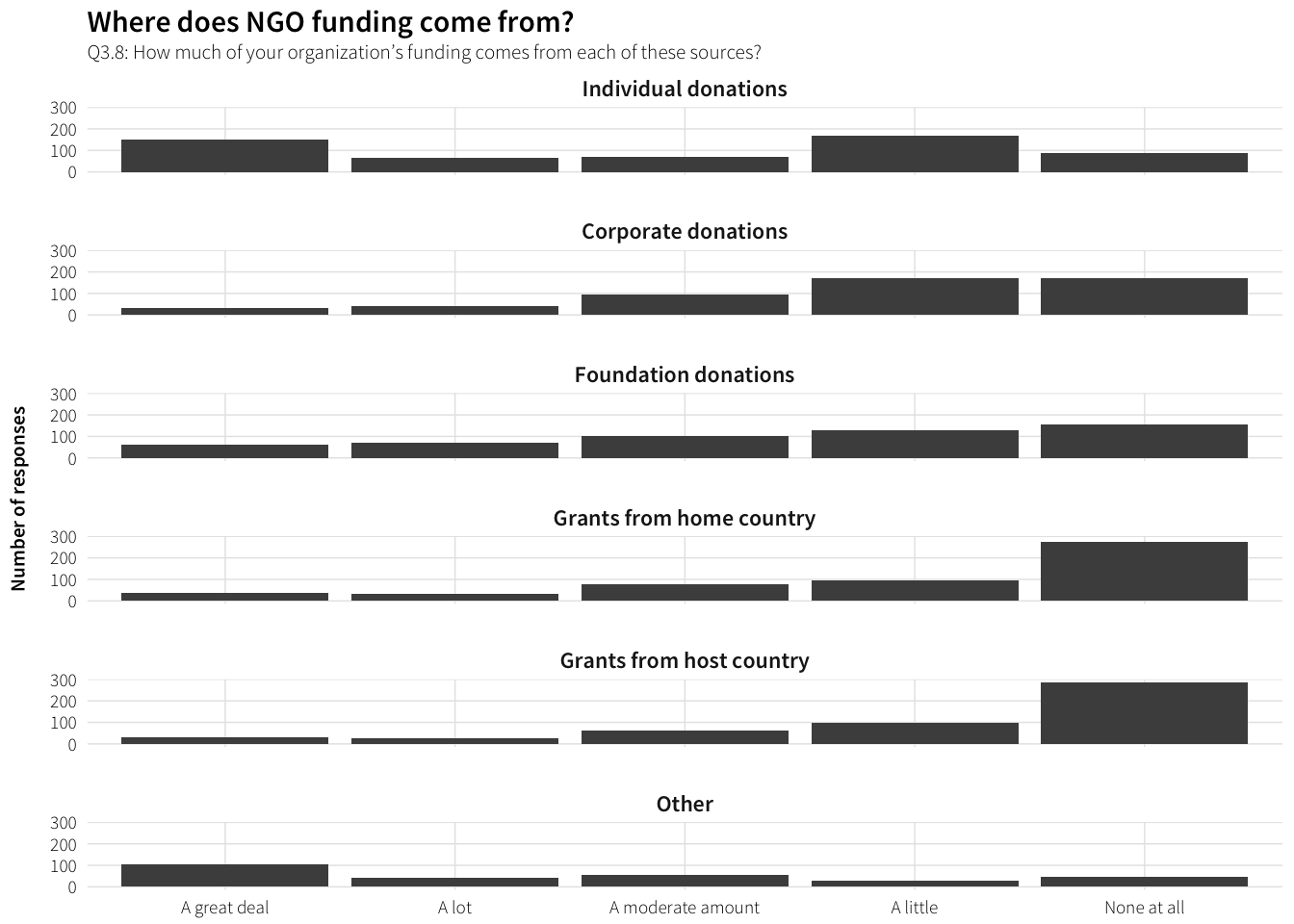What other sources of funding do NGOs use?

df.funding.other <- survey.orgs.clean %>%
filter(!is.na(Q3.8_other_TEXT)) %>%
arrange(Q3.8_other_TEXT) %>% select(Q3.8_other_TEXT)

datatable(df.funding.other)

The EU, churches, membership fees, etc.

## Deeper principles

### What is the NGO trying to accomplish?

Q3.9: In general, what would you say your organization is trying to accomplish?

# TODO: NLP/hand code these things
df.Q3.9 <- survey.orgs.clean %>%
filter(!is.na(Q3.9)) %>%
arrange(Q3.9) %>% select(Q3.9)

datatable(df.Q3.9)

### How are mission, vision, and values reflected in activities?

Q3.10: How is your organization’s mission, vision, and values reflected in these objectives?

df.Q3.10 <- survey.orgs.clean %>%
filter(!is.na(Q3.10)) %>%
arrange(Q3.10) %>% select(Q3.10)

datatable(df.Q3.10)

### Have these objectives changed?

Q3.11: Have these objectives changed at all in the last 10 years? If so, how?

df.Q3.11 <- survey.orgs.clean %>%
filter(!is.na(Q3.11)) %>%
arrange(Q3.11) %>% select(Q3.11)

datatable(df.Q3.11)

### Obstacles to objectives

Q3.12: What are the major obstacles, if any, to reaching your organization’s objectives?

df.Q3.12 <- survey.orgs.clean %>%
filter(!is.na(Q3.12)) %>%
arrange(Q3.12) %>% select(Q3.12)

datatable(df.Q3.12)

### Desired changes in goals and strategies

Q3.13: Are there any changes that you would like to see in your organization’s goals and strategies, now or in the future?

df.Q3.13 <- survey.orgs.clean %>%
filter(!is.na(Q3.13)) %>%
arrange(Q3.13) %>% select(Q3.13)

datatable(df.Q3.13)

## Government relations

### General questions

#### Time in country

df.time.country <- survey.countries.clean %>%
filter(!is.na(Q4.2)) %>%
group_by(Q4.2) %>%
summarise(num = n()) %>%
ungroup() %>%
mutate(Q4.2 = factor(Q4.2, levels=rev(levels(Q4.2)), ordered=TRUE))

plot.time.country <- ggplot(df.time.country, aes(x=num, y=Q4.2)) +
geom_barh(stat="identity") +
scale_x_continuous(expand=c(0, 0)) +
labs(x="Number of responses", y=NULL,
title="How long has the NGO worked in the country?",
subtitle="Q4.2: How long has your organization worked in target_country?") +
theme_ath()

plot.time.country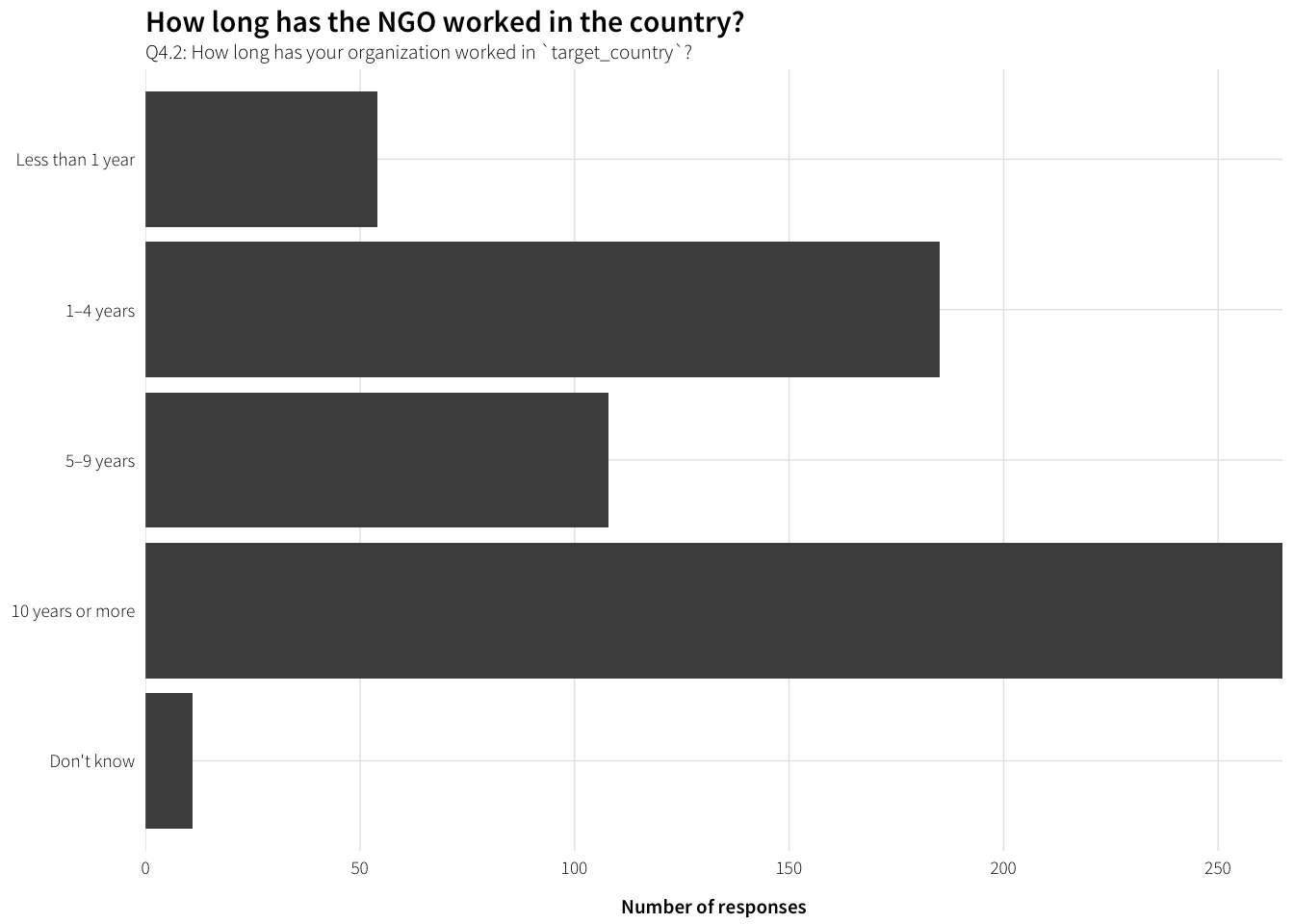#### Operations in country

df.operations <- survey.countries.clean %>%
unnest(Q4.3_value) %>%
group_by(Q4.3_value) %>%
summarise(num = n()) %>%
arrange(desc(num)) %>%
filter(!is.na(Q4.3_value)) %>%
mutate(operation = factor(Q4.3_value, levels=rev(Q4.3_value), ordered=TRUE))

# Add line breaks to labels
levels(df.operations$operation)[levels(df.operations$operation) == "Maintain a physical office staffed primarily by people from target_country"] <-
"Maintain a physical office staffed\nprimarily by people from target_country"
levels(df.operations$operation)[levels(df.operations$operation) == "Maintain a physical office staffed primarily by foreigners"] <-
"Maintain a physical office staffed\nprimarily by foreigners"

plot.operations <- ggplot(df.operations, aes(x=num, y=operation)) +
geom_barh(stat="identity") +
scale_x_continuous(expand=c(0, 0)) +
labs(x="Times selected", y=NULL,
title="How do NGOs work in the target country?",
subtitle="Q4.3: What does your organization do in target_country? (multiple answers allowed)") +
theme_ath()

plot.operations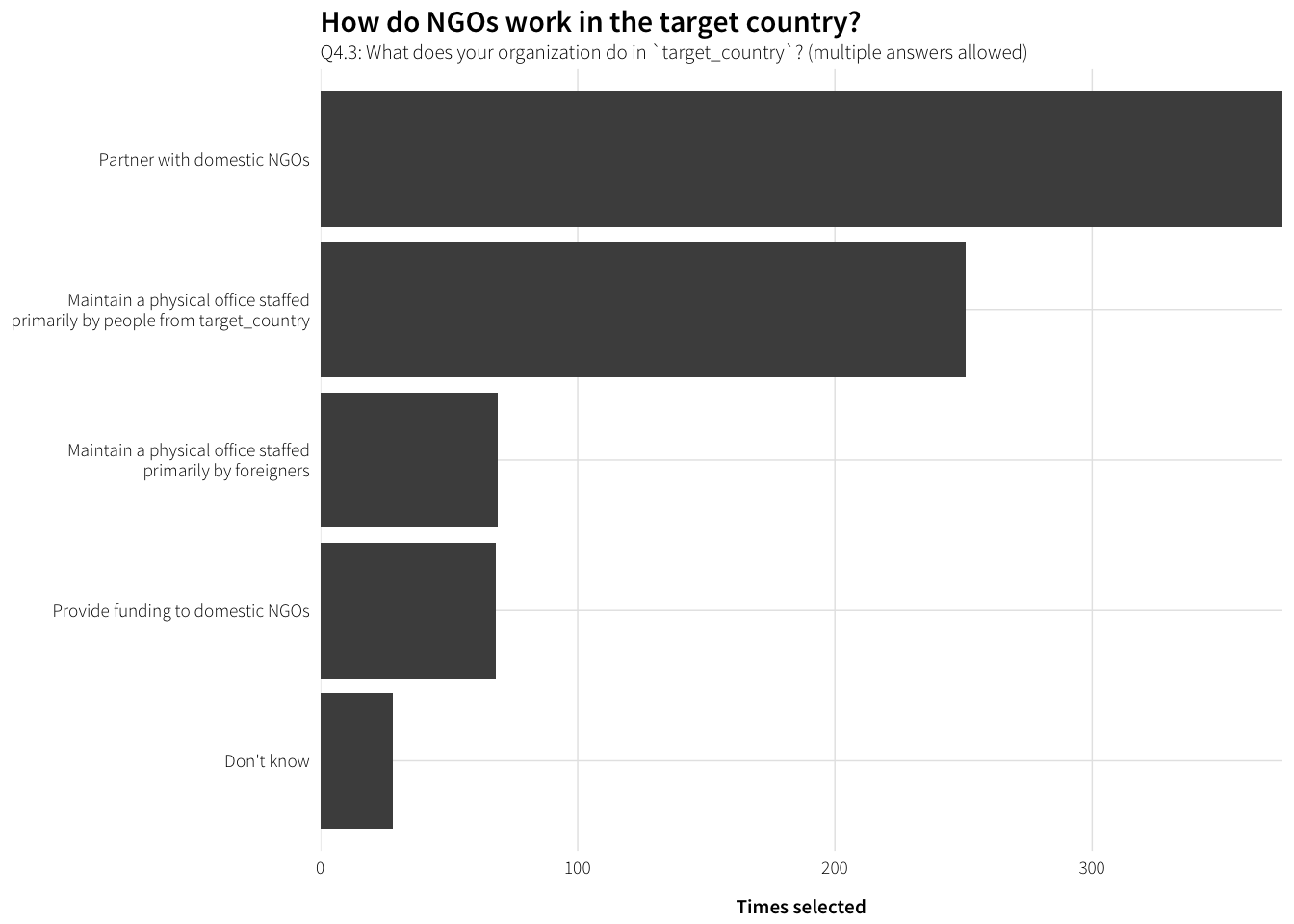#### Is the NGO registered?

df.registered <- survey.countries.clean %>%
filter(!is.na(Q4.4)) %>%
group_by(Q4.4) %>%
summarise(num = n()) %>%
ungroup() %>%
mutate(Q4.4 = factor(Q4.4, levels=rev(levels(Q4.4)), ordered=TRUE))

plot.registered <- ggplot(df.registered, aes(x=num, y=Q4.4)) +
geom_barh(stat="identity") +
scale_x_continuous(expand=c(0, 0)) +
labs(x="Number of responses", y=NULL,
title="Is the NGO registered?",
subtitle="Q4.4: Is your organization registered with the national government in target_country?") +
theme_ath()

plot.registered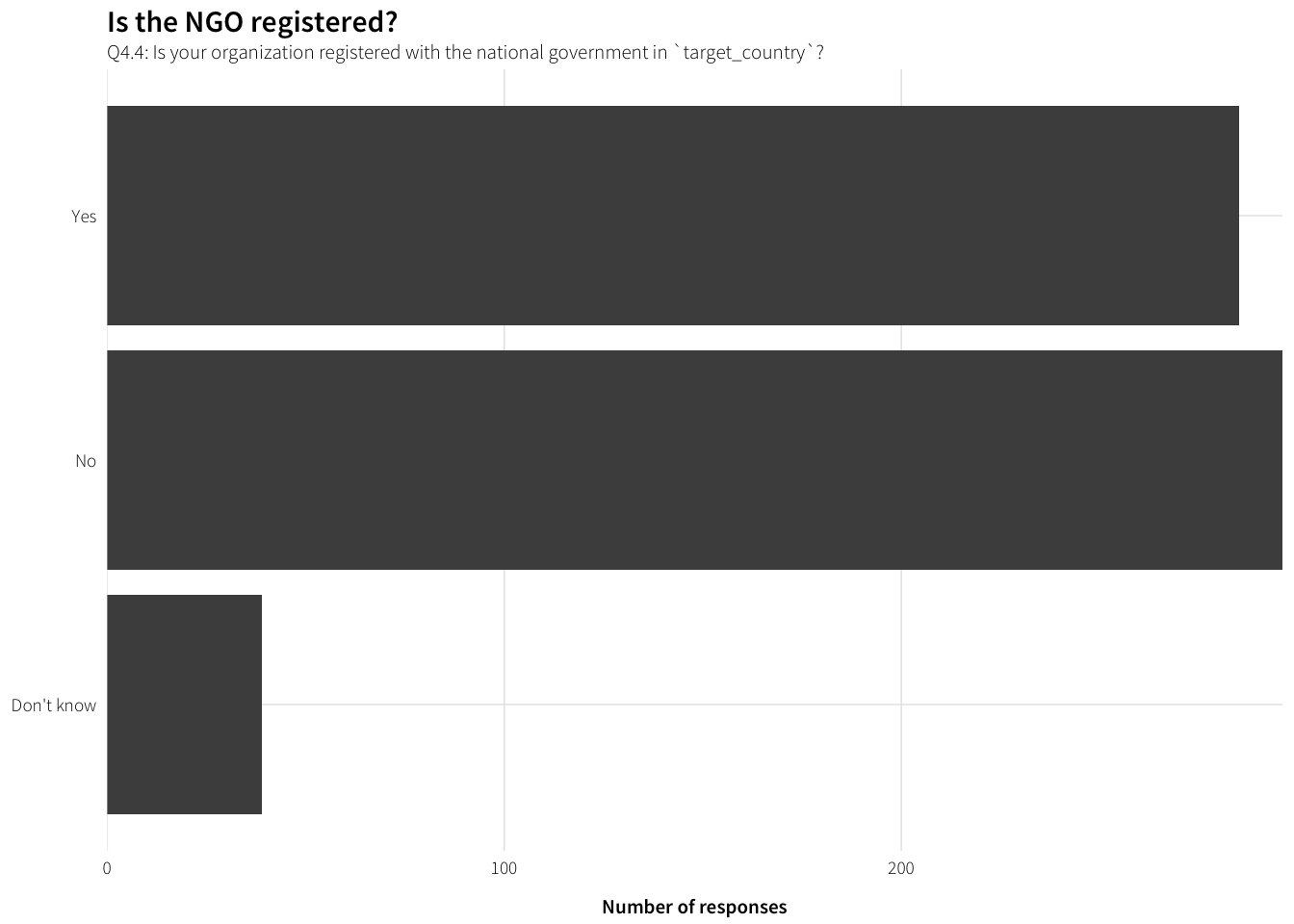## Contact with government

### Frequency of government contact

df.freq.contact <- survey.countries.clean %>%
filter(!is.na(Q4.5)) %>%
group_by(Q4.5) %>%
summarise(num = n()) %>%
ungroup() %>%
mutate(Q4.5 = factor(Q4.5, levels=rev(levels(Q4.5)), ordered=TRUE))

plot.freq.contact <- ggplot(df.freq.contact, aes(x=num, y=Q4.5)) +
geom_barh(stat="identity") +
scale_x_continuous(expand=c(0, 0)) +
labs(x="Number of responses", y=NULL,
title="How often does the NGO contact government?",
subtitle="Q4.5: About how often does your organization have contact with\ngovernment or party officials in target_country?") +
theme_ath()

plot.freq.contact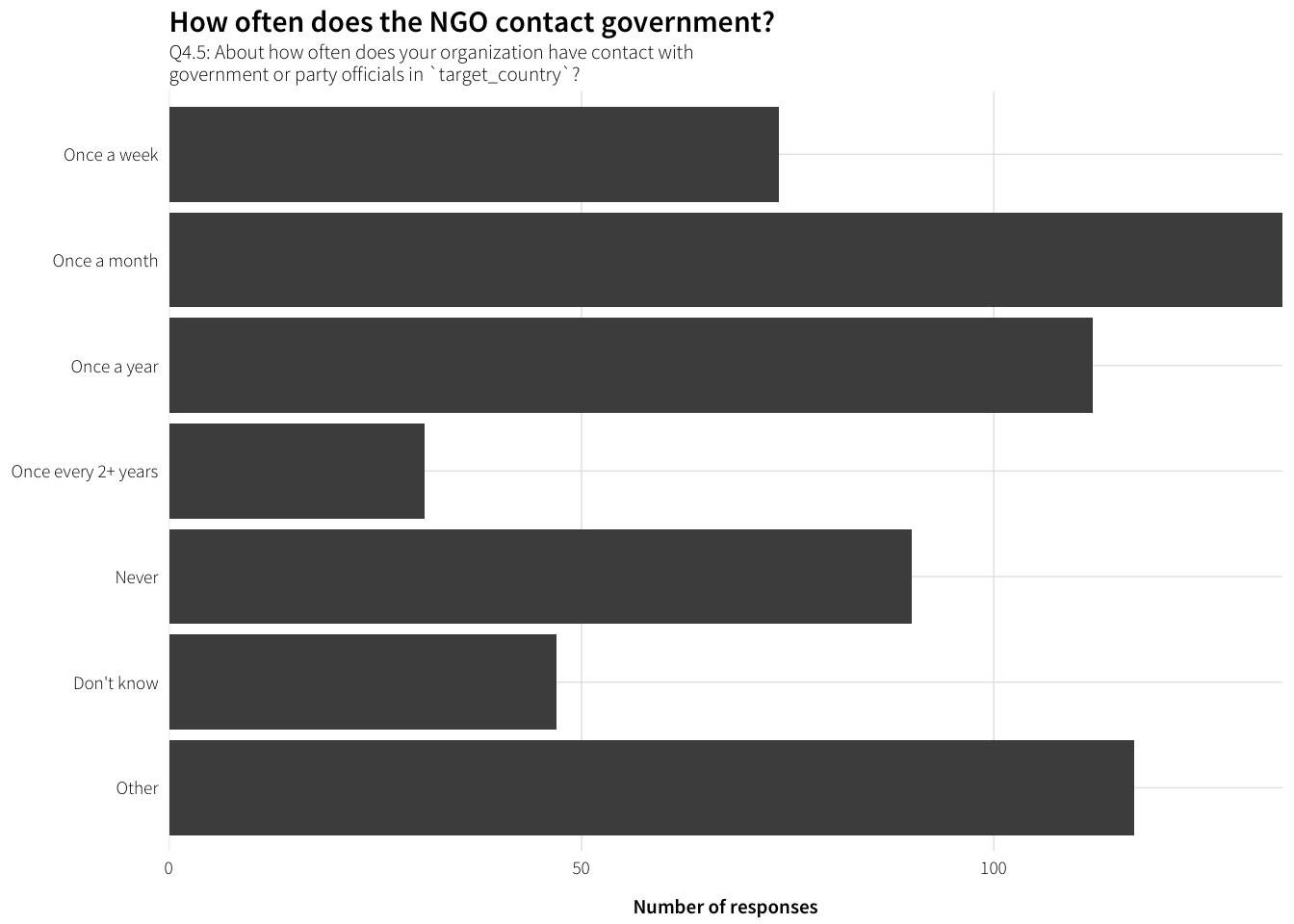What other kinds of frequency do people report?

df.freq.contact.other <- survey.countries.clean %>%
filter(!is.na(Q4.5_TEXT)) %>%
mutate(other.freq = str_to_title(Q4.5_TEXT)) %>%
group_by(other.freq) %>%
summarise(num = n()) %>%
arrange(desc(num))

datatable(df.freq.contact.other)

With all the other options cleaned up

df.freq.contact.clean <- survey.countries.clean %>%
filter(!is.na(Q4.5.clean)) %>%
group_by(Q4.5.clean) %>%
summarise(num = n()) %>%
ungroup() %>%
mutate(Q4.5.clean = factor(Q4.5.clean, levels=rev(levels(Q4.5.clean)),
ordered=TRUE))

plot.freq.contact.clean <- ggplot(df.freq.contact.clean,
aes(x=num, y=Q4.5.clean)) +
geom_barh(stat="identity") +
scale_x_continuous(expand=c(0, 0)) +
labs(x="Number of responses", y=NULL,
title="How often does the NGO contact government?",
subtitle="Q4.5: About how often does your organization have contact with\ngovernment or party officials in target_country?") +
theme_ath()

plot.freq.contact.clean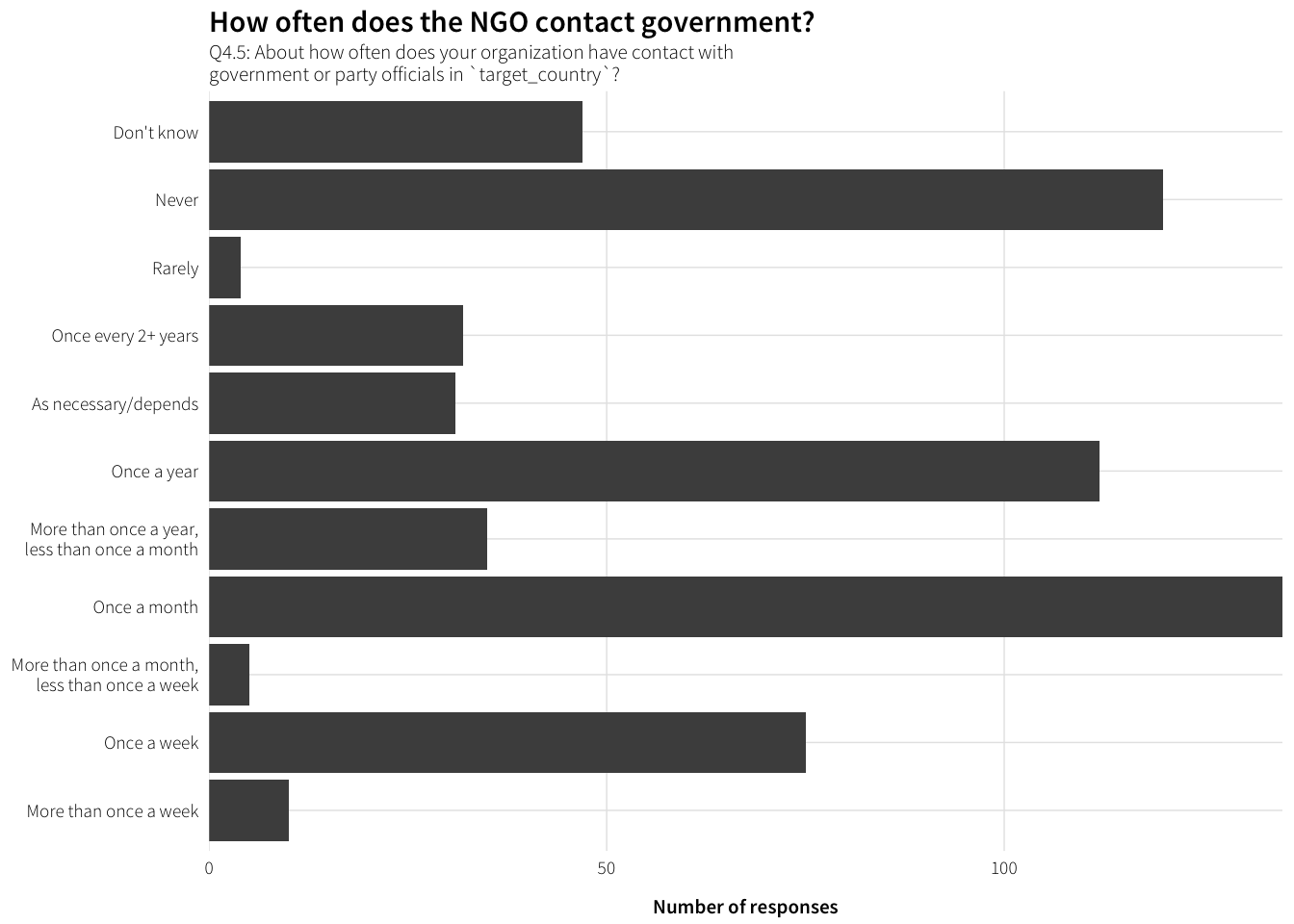### Frequency of reporting to government

df.freq.report <- survey.countries.clean %>%
filter(!is.na(Q4.8)) %>%
group_by(Q4.8) %>%
summarise(num = n()) %>%
ungroup() %>%
mutate(Q4.8 = factor(Q4.8, levels=rev(levels(Q4.8)), ordered=TRUE))

plot.freq.report <- ggplot(df.freq.report, aes(x=num, y=Q4.8)) +
geom_barh(stat="identity") +
scale_x_continuous(expand=c(0, 0)) +
labs(x="Number of responses", y=NULL,
title="How often does the NGO report to government?",
subtitle="Q4.8: How often is your organization required to report to the government of  target_country?") +
theme_ath()

plot.freq.report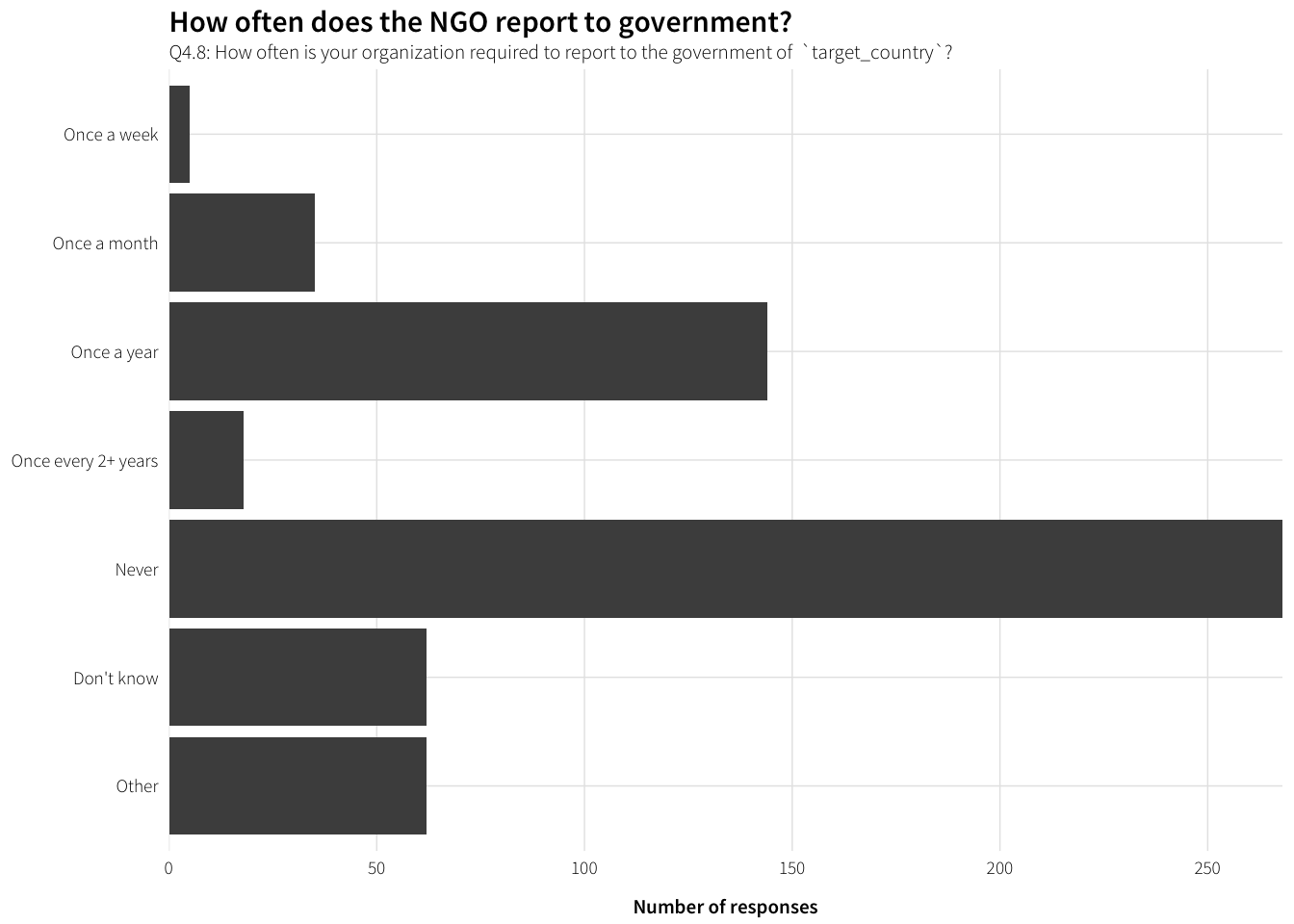What other kinds of frequency do people report?

df.freq.report.other <- survey.countries.clean %>%
filter(!is.na(Q4.8_TEXT)) %>%
select(Q4.8_TEXT) %>%
arrange(Q4.8_TEXT)

datatable(df.freq.report.other)

With all the other options cleaned up

df.freq.report.clean <- survey.countries.clean %>%
filter(!is.na(Q4.8.clean)) %>%
group_by(Q4.8.clean) %>%
summarise(num = n()) %>%
ungroup() %>%
mutate(Q4.8.clean = factor(Q4.8.clean, levels=rev(levels(Q4.8.clean)),
ordered=TRUE))

plot.freq.report.clean <- ggplot(df.freq.report.clean,
aes(x=num, y=Q4.8.clean)) +
geom_barh(stat="identity") +
scale_x_continuous(expand=c(0, 0)) +
labs(x="Number of responses", y=NULL,
title="How often does the NGO report to government?",
subtitle="Q4.8: How often is your organization required to report to the government of  target_country?") +
theme_ath()

plot.freq.report.clean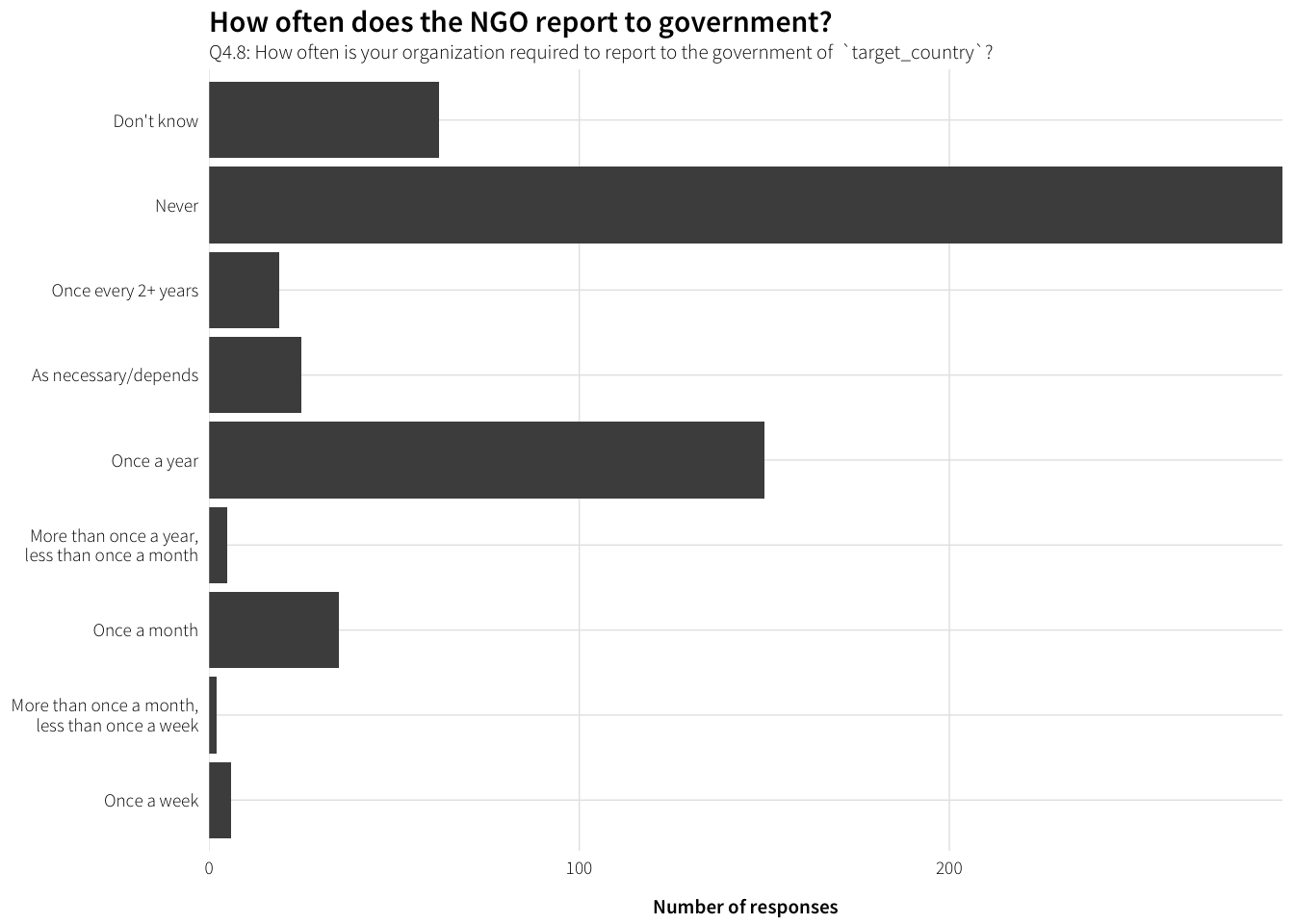### Kinds of government officials NGOs have contact with

df.officials.contact <- survey.countries.clean %>%
unnest(Q4.6_value) %>%
group_by(Q4.6_value) %>%
summarise(num = n()) %>%
arrange(desc(num)) %>%
filter(!is.na(Q4.6_value)) %>%
mutate(official = factor(Q4.6_value, levels=rev(Q4.6_value), ordered=TRUE))

plot.officials.contact <- ggplot(df.officials.contact, aes(x=num, y=official)) +
geom_barh(stat="identity") +
scale_x_continuous(expand=c(0, 0)) +
labs(x="Times selected", y=NULL,
title="Which officials do NGOs report to?",
subtitle="Q4.6: What kind of government officials does your organization have contact with? (multiple answers allowed)") +
theme_ath()

plot.officials.contact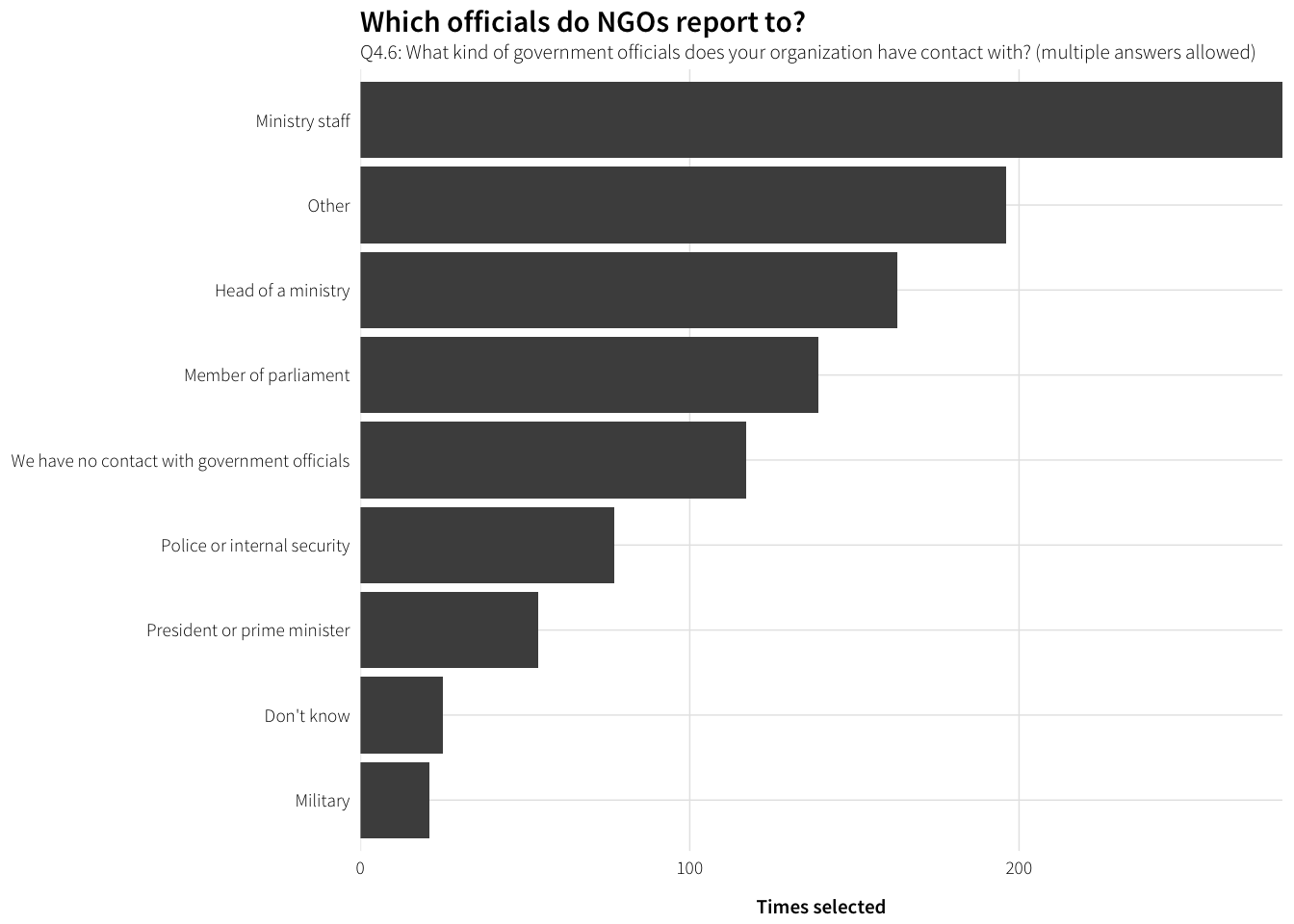And the others?

df.officials.contact.other <- survey.countries.clean %>%
filter(!is.na(Q4.6_other_TEXT)) %>%
mutate(official = str_to_title(Q4.6_other_TEXT)) %>%
group_by(official) %>%
summarise(num = n()) %>%
arrange(desc(num))

datatable(df.officials.contact.other)

### Officials reported to the most

df.officials.contact.most <- survey.countries.clean %>%
group_by(Q4.7) %>%
summarise(num = n()) %>%
arrange(desc(num)) %>%
filter(!is.na(Q4.7)) %>%
mutate(issue = factor(Q4.7, levels=rev(Q4.7), ordered=TRUE))

plot.officials.contact.most <- ggplot(df.officials.contact.most,
aes(x=num, y=issue)) +
geom_barh(stat="identity") +
scale_x_continuous(expand=c(0, 0)) +
labs(x="Times selected", y=NULL,
title="Which officials do NGOs report to the most?",
subtitle="Q4.7: What kind of government officials does your organization have contact with most often?") +
theme_ath()

plot.officials.contact.most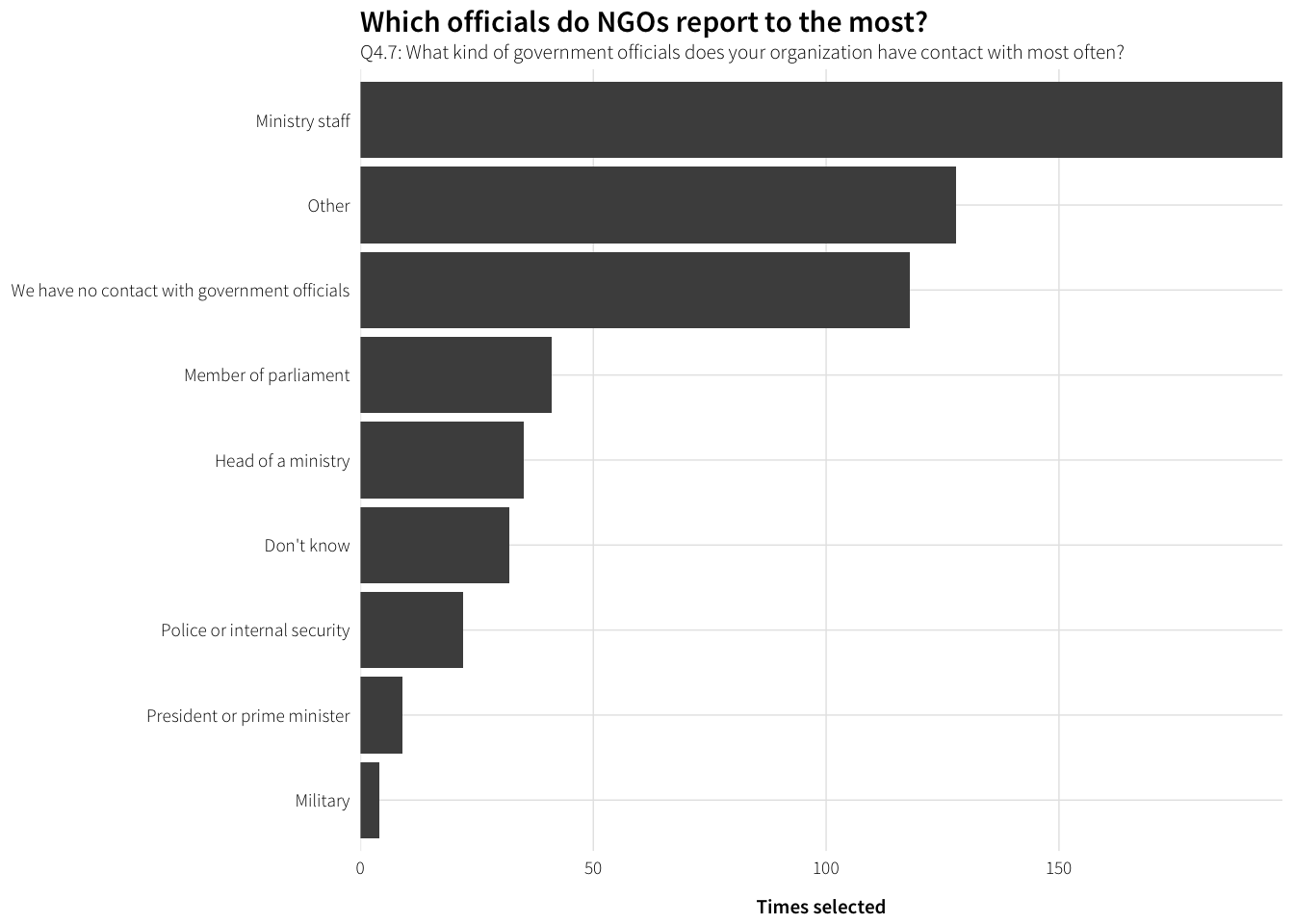Who are the others?

df.officials.contact.most.other <- survey.countries.clean %>%
filter(!is.na(Q4.7_TEXT)) %>%
rename(official = Q4.7_TEXT) %>%
group_by(official) %>%
summarise(num = n()) %>%
arrange(desc(num))

datatable(df.officials.contact.most.other)

### Are members of the government involved in work?

df.govt.involved <- survey.countries.clean %>%
filter(!is.na(Q4.9)) %>%
group_by(Q4.9) %>%
summarise(num = n()) %>%
ungroup() %>%
mutate(Q4.9 = factor(Q4.9, levels=rev(levels(Q4.9)), ordered=TRUE))

plot.govt.involved <- ggplot(df.govt.involved, aes(x=num, y=Q4.9)) +
geom_barh(stat="identity") +
scale_x_continuous(expand=c(0, 0)) +
labs(x="Number of responses", y=NULL,
title="Is the government involved in the NGO's work?",
subtitle="Q4.9: Are members of the government or ruling party of target_country involved in your work?") +
theme_ath()

plot.govt.involved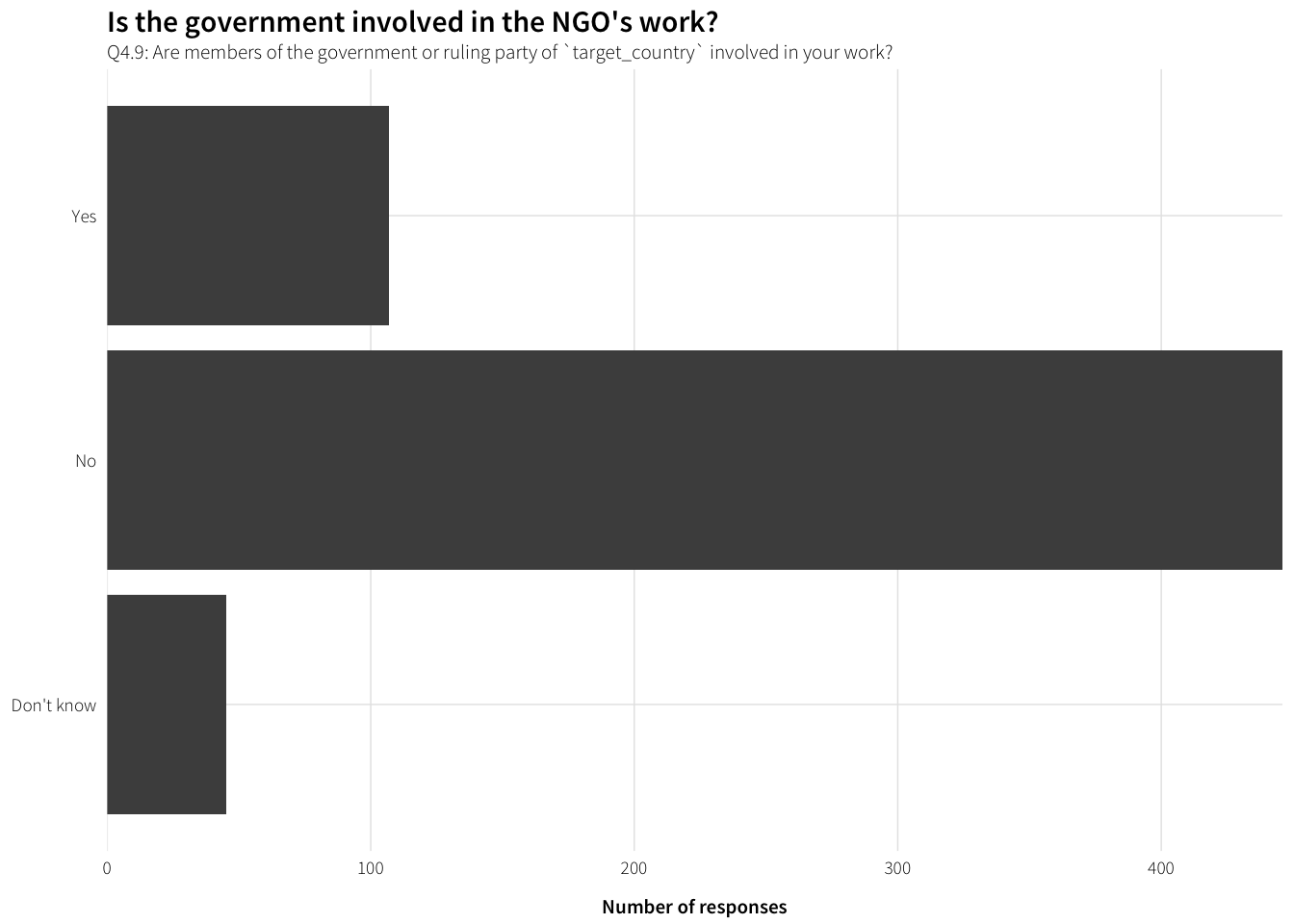### How is government involved in work?

Q4.10: How is the government of target_country involved in your work?

df.Q4.10 <- survey.countries.clean %>%
filter(!is.na(Q4.10)) %>%
arrange(Q4.10) %>% select(Q4.10)

datatable(df.Q4.10)

## Relationship with government

### Positivity of relationship

df.govt.positivity <- survey.countries.clean %>%
filter(!is.na(Q4.11)) %>%
group_by(Q4.11) %>%
summarise(num = n()) %>%
ungroup() %>%
mutate(Q4.11 = factor(Q4.11, levels=rev(levels(Q4.11)), ordered=TRUE))

plot.govt.positivity <- ggplot(df.govt.positivity, aes(x=num, y=Q4.11)) +
geom_barh(stat="identity") +
scale_x_continuous(expand=c(0, 0)) +
labs(x="Number of responses", y=NULL,
title="How is the NGO's relationship with the government?",
subtitle="Q4.11: How would you characterize your organization’s relationship with the government of target_country?") +
theme_ath()

plot.govt.positivity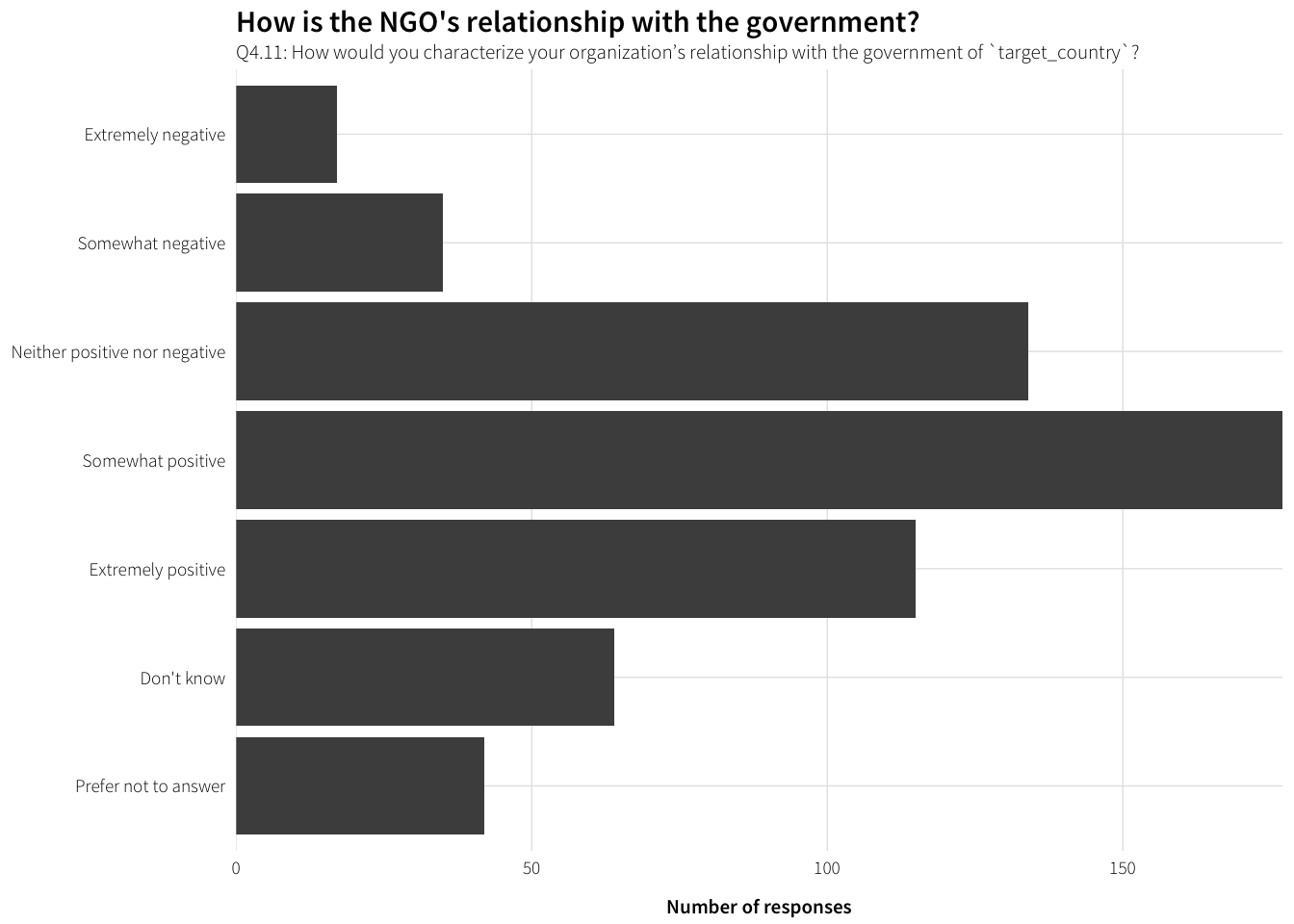### Description of relationship

Q4.12: Briefly describe your organization’s relationship with the government of target_country:

df.Q4.12 <- survey.countries.clean %>%
filter(!is.na(Q4.12)) %>%
arrange(Q4.12) %>% select(Q4.12)

datatable(df.Q4.12)

## NGO regulations and restrictions

### Familiarity with regulations

df.reg.familiarity <- survey.countries.clean %>%
filter(!is.na(Q4.13)) %>%
group_by(Q4.13) %>%
summarise(num = n()) %>%
ungroup() %>%
mutate(Q4.13 = factor(Q4.13, levels=rev(levels(Q4.13)), ordered=TRUE))

plot.reg.familiarity <- ggplot(df.reg.familiarity, aes(x=num, y=Q4.13)) +
geom_barh(stat="identity") +
scale_x_continuous(expand=c(0, 0)) +
labs(x="Number of responses", y=NULL,
title="How familiar are NGOs with regulations?",
subtitle="Q4.13: How familiar is your organization with regulations for\ninternational nongovernmental organizations (NGOs) in target_country?") +
theme_ath()

plot.reg.familiarity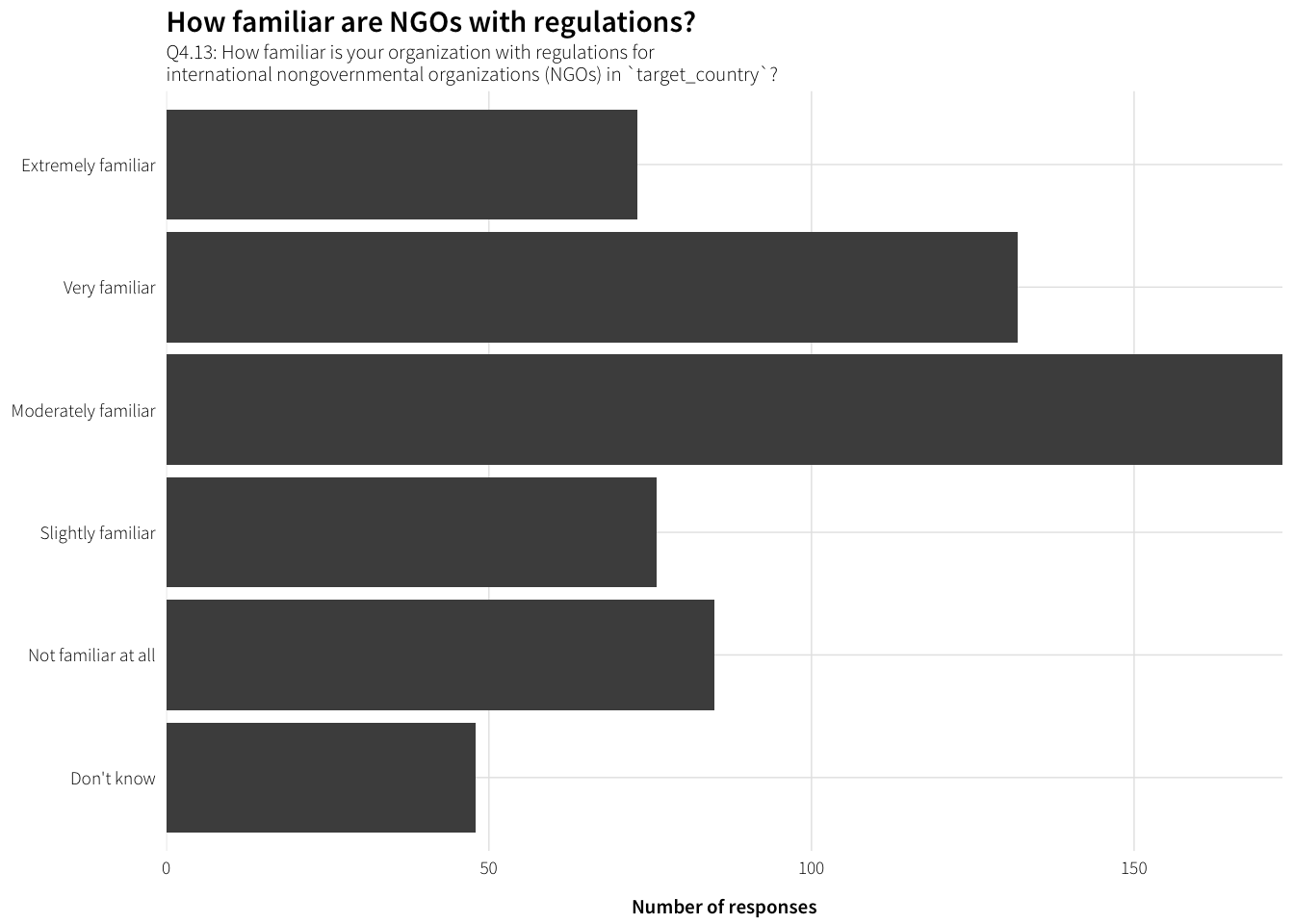### Frequency of regulation change

df.reg.change <- survey.countries.clean %>%
filter(!is.na(Q4.14)) %>%
group_by(Q4.14) %>%
summarise(num = n()) %>%
ungroup() %>%
mutate(Q4.14 = factor(Q4.14, levels=rev(levels(Q4.14)), ordered=TRUE))

plot.reg.change <- ggplot(df.reg.change, aes(x=num, y=Q4.14)) +
geom_barh(stat="identity") +
scale_x_continuous(expand=c(0, 0)) +
labs(x="Number of responses", y=NULL,
title="How often do NGO regulations change?",
subtitle="Q4.14: How often do regulations for international NGOs in target_country change?") +
theme_ath()

plot.reg.change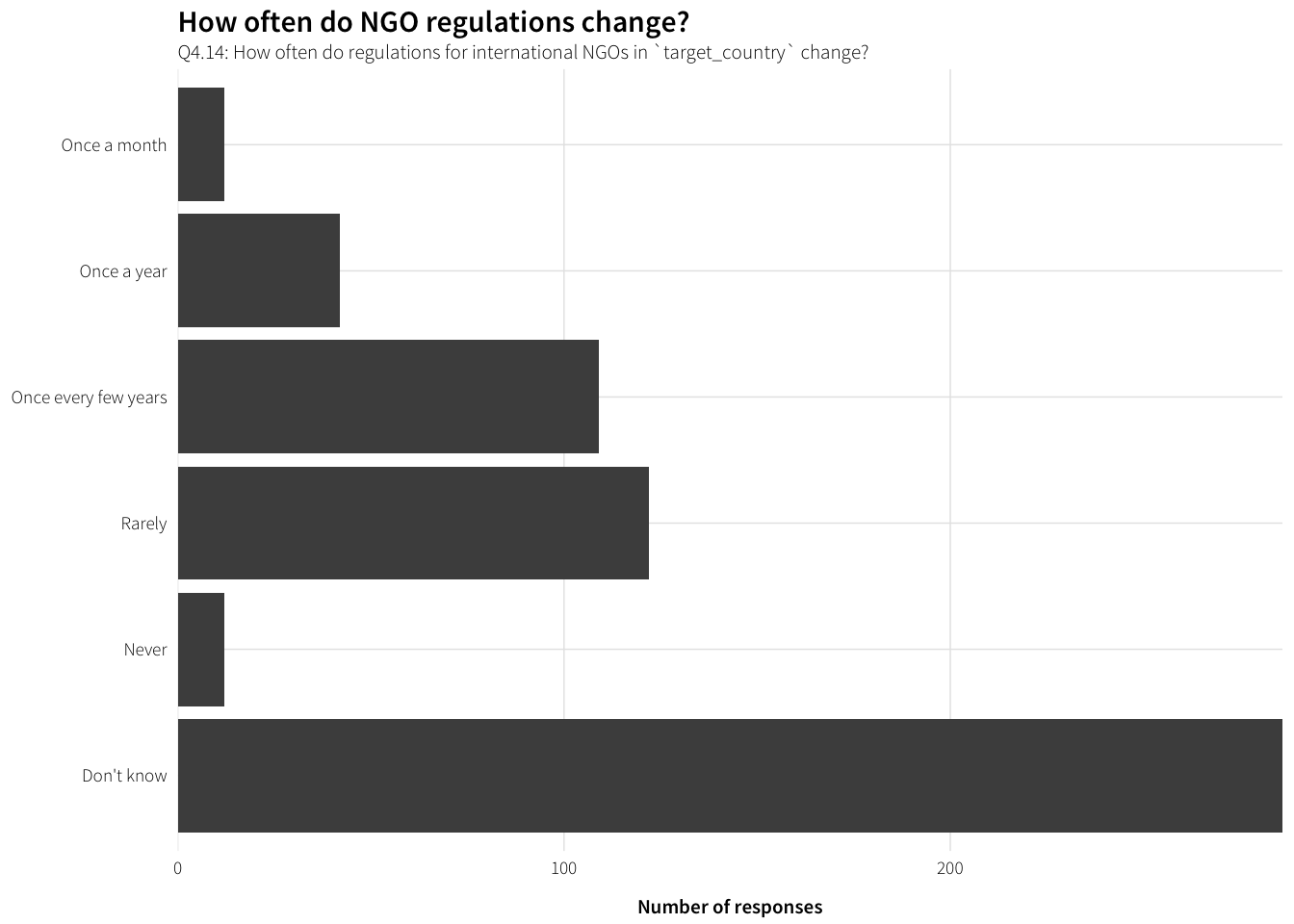### How do NGOs find out about changes?

df.change.how <- survey.countries.clean %>%
unnest(Q4.15_value) %>%
group_by(Q4.15_value) %>%
summarise(num = n()) %>%
arrange(desc(num)) %>%
filter(!is.na(Q4.15_value)) %>%
mutate(how = factor(Q4.15_value, levels=rev(Q4.15_value), ordered=TRUE))

levels(df.change.how$how)[levels(df.change.how$how) == "Newspapers, television, and other media"] <-
"Newspapers, television,\nand other media"

plot.change.how <- ggplot(df.change.how, aes(x=num, y=how)) +
geom_barh(stat="identity") +
scale_x_continuous(expand=c(0, 0)) +
labs(x="Times selected", y=NULL,
title="How do NGOs find out about changes to regulations?",
subtitle="Q4.15: How does your organization find out about changes to\nNGO regulations in target_country? (multiple answers allowed)") +
theme_ath()

plot.change.how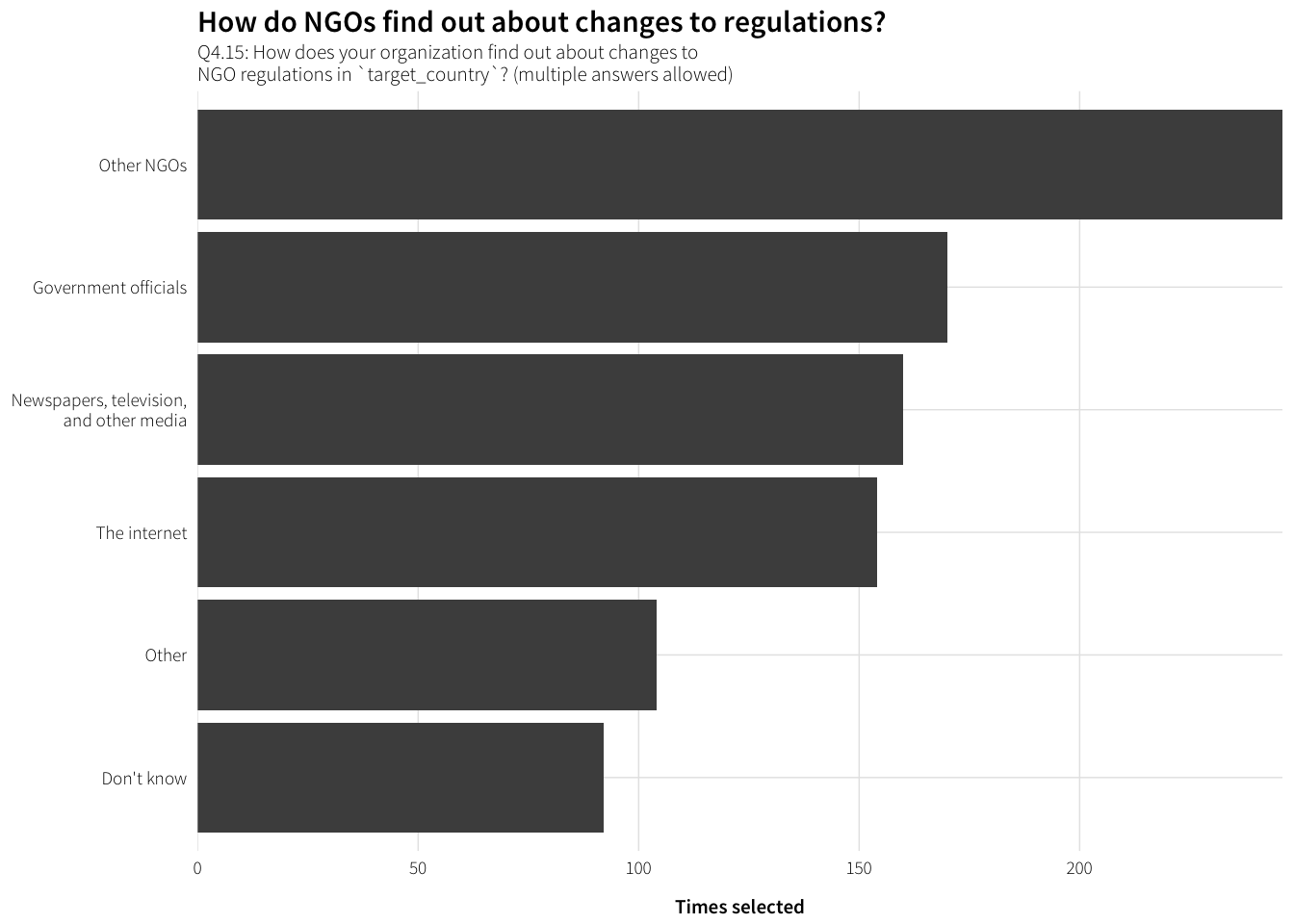What are the other ways?

df.change.how.other <- survey.countries.clean %>%
filter(!is.na(Q4.15_other_TEXT)) %>%
select(Q4.15_other_TEXT) %>%
arrange(Q4.15_other_TEXT)

datatable(df.change.how.other)

### How are NGOs affected by regulations?

labels.reg.effects <- data_frame(levels=c("registration", "operations", "speech",
"communications", "assembly", "resources"),
labels=c("Regulations regarding registration",
"Regulations regarding operations",
"Regulations regarding communication and cooperation",
"Regulations regarding assembly",
"Regulations regarding resources"))

df.reg.effects <- survey.countries.clean %>%
select(dplyr::contains("Q4.16"), -dplyr::contains("TEXT")) %>%
gather(question, response) %>%
mutate(question = str_replace(question, "Q4\\.16_", ""),
question = factor(question, levels=labels.reg.effects$levels, labels=labels.reg.effects$labels, ordered=TRUE)) %>%
filter(!(response %in% c("Don't know", "Not applicable"))) %>%
group_by(question, response) %>%
summarise(num = n()) %>%
ungroup() %>%
mutate(response = factor(response,
levels=levels(survey.countries.clean$Q4.16_speech), ordered=TRUE)) plot.reg.effects <- ggplot(df.reg.effects, aes(y=num, x=response)) + geom_bar(stat="identity") + labs(y="Number of responses", x=NULL, title="How are NGOs affected by regulations?", subtitle="Q4.16: How is your organization affected by the following types\nof legal regulations for international NGOs in target_country?") + facet_wrap(~ question, ncol=1) + theme_ath() plot.reg.effects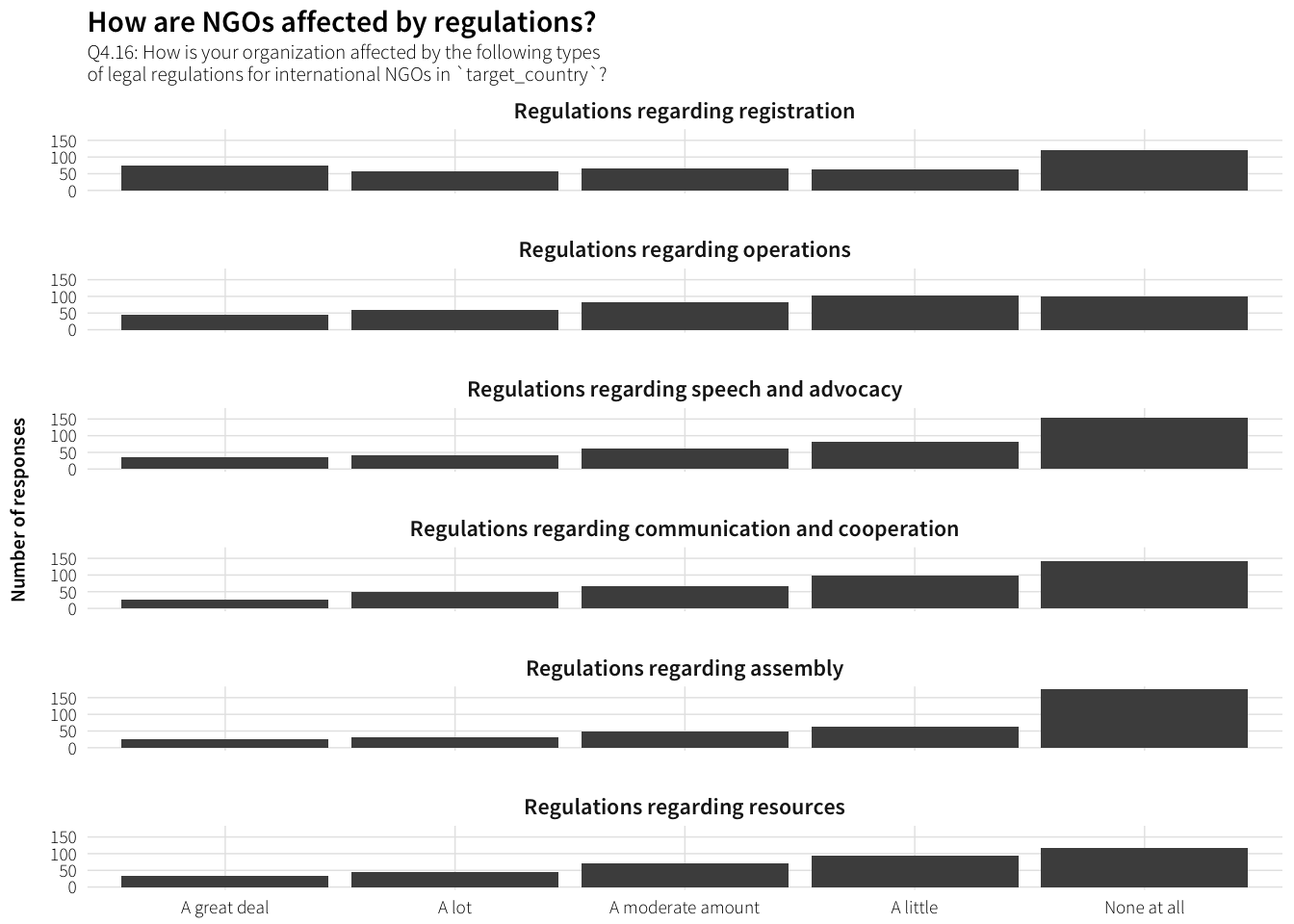#### Regulations regarding registration Q4.16a: Please describe how your organization is affected by regulations regarding registration. df.Q4.16.registration <- survey.countries.clean %>% filter(!is.na(Q4.16_registration_TEXT)) %>% arrange(Q4.16_registration_TEXT) %>% select(Q4.16_registration_TEXT) datatable(df.Q4.16.registration) #### Regulations regarding operations Q4.16b: Please describe how your organization is affected by regulations regarding operations. df.Q4.16.operations <- survey.countries.clean %>% filter(!is.na(Q4.16_operations_TEXT)) %>% arrange(Q4.16_operations_TEXT) %>% select(Q4.16_operations_TEXT) datatable(df.Q4.16.operations) #### Regulations regarding speech and advocacy Q4.16c: Please describe how your organization is affected by regulations regarding speech and advocacy. df.Q4.16.speech <- survey.countries.clean %>% filter(!is.na(Q4.16_speech_TEXT)) %>% arrange(Q4.16_speech_TEXT) %>% select(Q4.16_speech_TEXT) datatable(df.Q4.16.speech) #### Regulations regarding communication and cooperation Q4.16d: Please describe how your organization is affected by regulations regarding communication and cooperation. df.Q4.16.communications <- survey.countries.clean %>% filter(!is.na(Q4.16_communications_TEXT)) %>% arrange(Q4.16_communications_TEXT) %>% select(Q4.16_communications_TEXT) datatable(df.Q4.16.communications) #### Regulations regarding assembly Q4.16e: Please describe how your organization is affected by regulations regarding assembly. df.Q4.16.assembly <- survey.countries.clean %>% filter(!is.na(Q4.16_assembly_TEXT)) %>% arrange(Q4.16_assembly_TEXT) %>% select(Q4.16_assembly_TEXT) datatable(df.Q4.16.assembly) #### Regulations regarding resources Q4.16f: Please describe how your organization is affected by regulations regarding resources. df.Q4.16.resources <- survey.countries.clean %>% filter(!is.na(Q4.16_resources_TEXT)) %>% arrange(Q4.16_resources_TEXT) %>% select(Q4.16_resources_TEXT) datatable(df.Q4.16.resources) ### How are NGOs affected by regulations in general? df.reg.effect.general <- survey.countries.clean %>% filter(!is.na(Q4.17)) %>% group_by(Q4.17) %>% summarise(num = n()) %>% ungroup() %>% mutate(Q4.17 = factor(Q4.17, levels=rev(levels(Q4.17)), ordered=TRUE)) plot.reg.effect.general <- ggplot(df.reg.effect.general, aes(x=num, y=Q4.17)) + geom_barh(stat="identity") + scale_x_continuous(expand=c(0, 0)) + labs(x="Number of responses", y=NULL, title="How are NGOs affected by regulations in general?", subtitle="Q4.17: Overall, how is your organization's work affected by government regulations in target_country?") + theme_ath() plot.reg.effect.general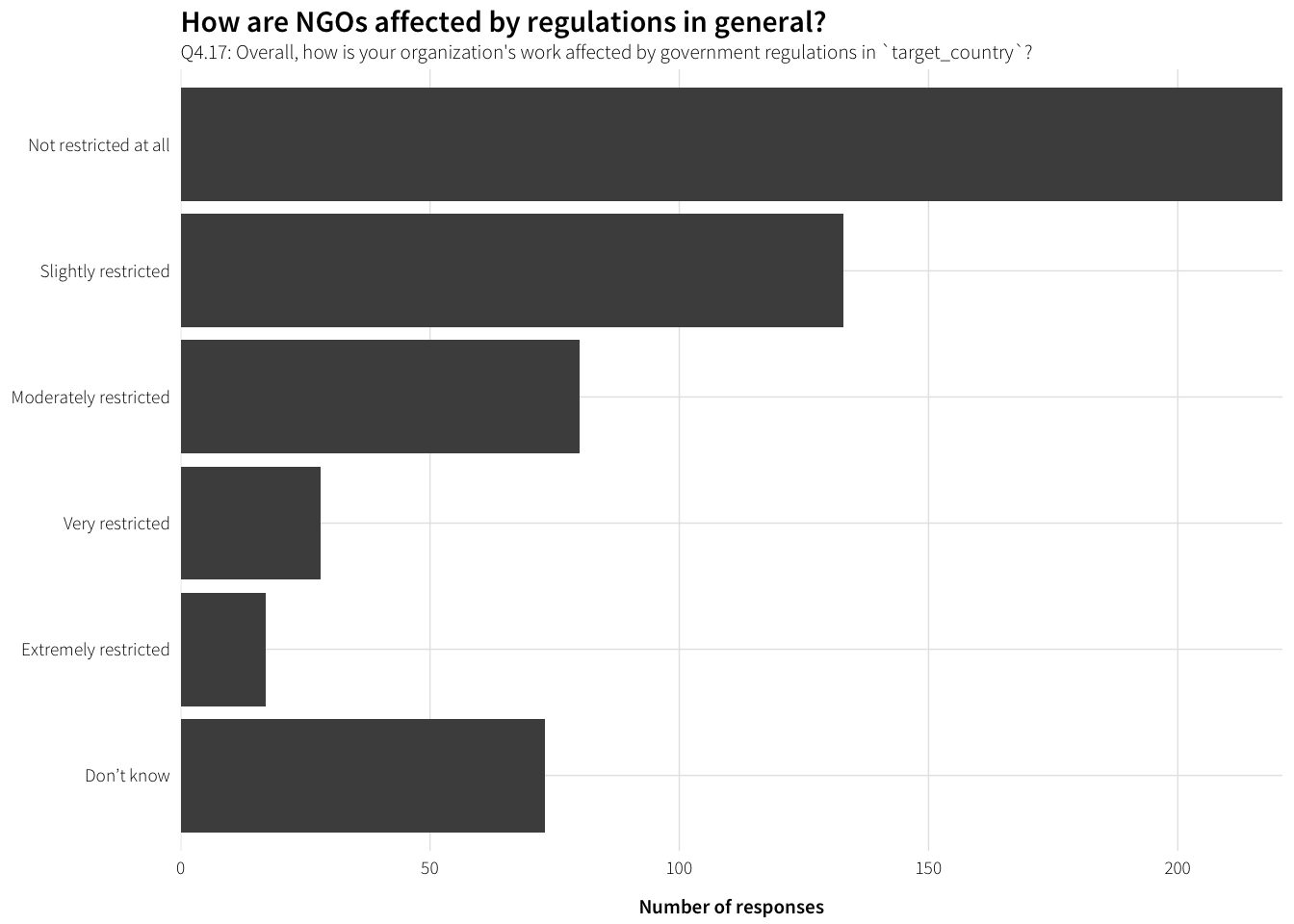### Effect of regulations on ability to pursue mission Q4.18: How do the local laws and regulations in target_country affect your organization’s ability to pursue its mission? df.Q4.18 <- survey.countries.clean %>% filter(!is.na(Q4.18)) %>% arrange(Q4.18) %>% select(Q4.18) datatable(df.Q4.18) ## Responses to regulations ### Have NGOs changed programming? df.change.programming <- survey.countries.clean %>% filter(!is.na(Q4.19)) %>% group_by(Q4.19) %>% summarise(num = n()) %>% ungroup() %>% mutate(Q4.19 = factor(Q4.19, levels=rev(levels(Q4.19)), ordered=TRUE)) plot.change.programming <- ggplot(df.change.programming, aes(x=num, y=Q4.19)) + geom_barh(stat="identity") + scale_x_continuous(expand=c(0, 0)) + labs(x="Number of responses", y=NULL, title="Have NGOs changed their mix of programming?", subtitle="Q4.19: Over the last 10 years, has your organization changed its mix of programming in target_country?") + theme_ath() plot.change.programming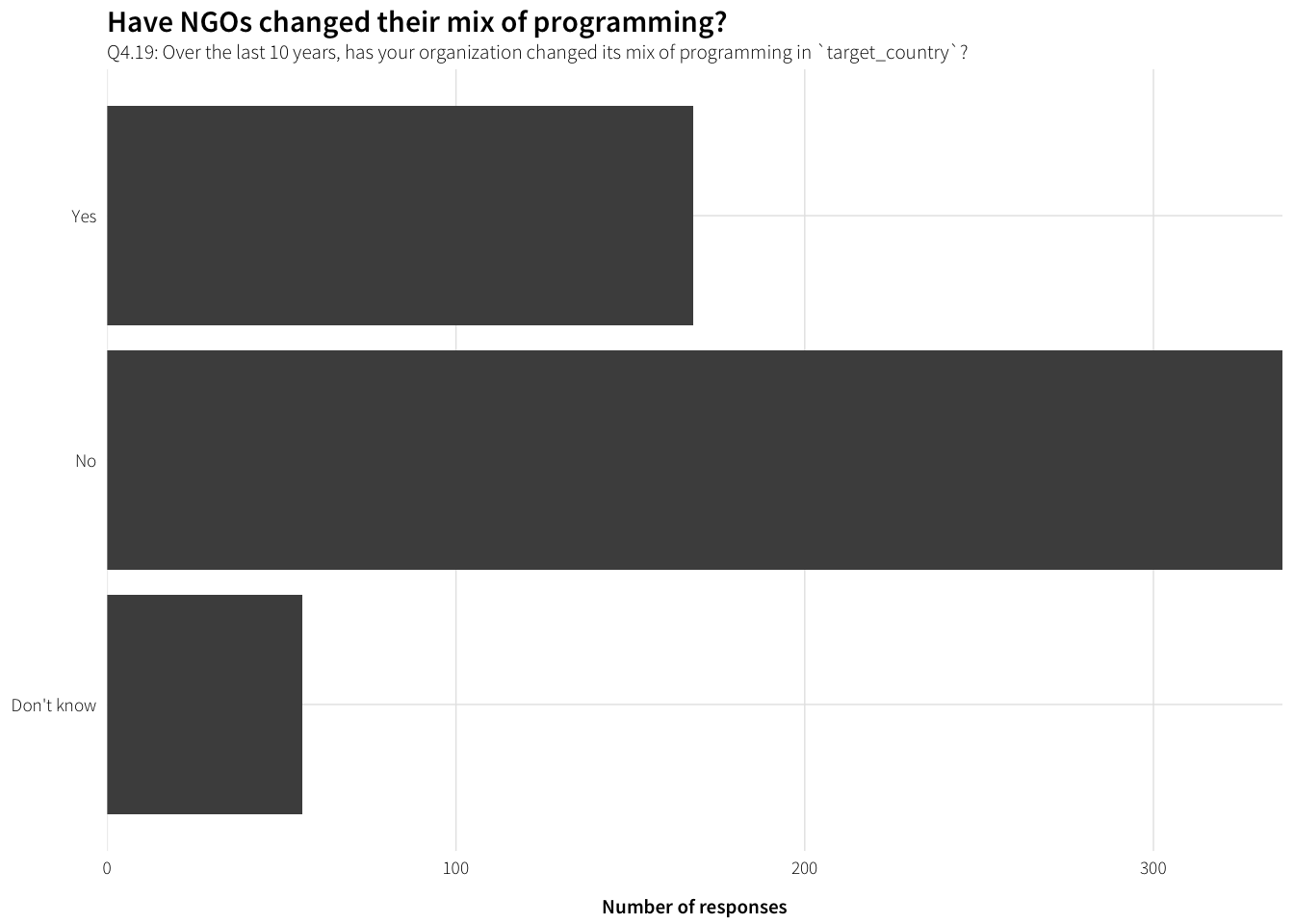How so? Q4.20: How has your organization’s mix of programming changed in target_country? df.Q4.20 <- survey.countries.clean %>% filter(!is.na(Q4.20)) %>% arrange(Q4.20) %>% select(Q4.20) datatable(df.Q4.20) ### Changes in response to regulations labels.changes <- data_frame(levels=c("funding", "issues", "comm_govt", "comm_donors", "locations", "country_office", "local_staff", "foreign_staff"), labels=c("Changed sources of funding", "Changed issues worked on", "Changed communication with the government", "Changed communication with donors", "Changed locations worked in", "Changed location country office", "Used more local staff and/or volunteers", "Used more foreign staff and/or volunteers")) df.reg.changes.response <- survey.countries.clean %>% select(dplyr::contains("Q4.21"), -dplyr::contains("TEXT")) %>% gather(question, response) %>% mutate(question = str_replace(question, "Q4\\.21_", ""), question = factor(question, levels=labels.changes$levels,
labels=labels.changes$labels, ordered=TRUE)) %>% filter(!(response %in% c("Don't know", "Not applicable"))) %>% group_by(question, response) %>% summarise(num = n()) %>% ungroup() %>% mutate(response = factor(response, levels=levels(survey.countries.clean$Q4.21_funding),
ordered=TRUE))

plot.reg.changes.response <- ggplot(df.reg.changes.response,
aes(y=num, x=response)) +
geom_bar(stat="identity") +
labs(y="Number of responses", x=NULL,
title="How have NGOs changed in response to regulations?",
subtitle="Q4.21: Has your organization done any of the following in response to changes in government regulations in target_country?") +
facet_wrap(~ question, ncol=2) +
theme_ath()

plot.reg.changes.response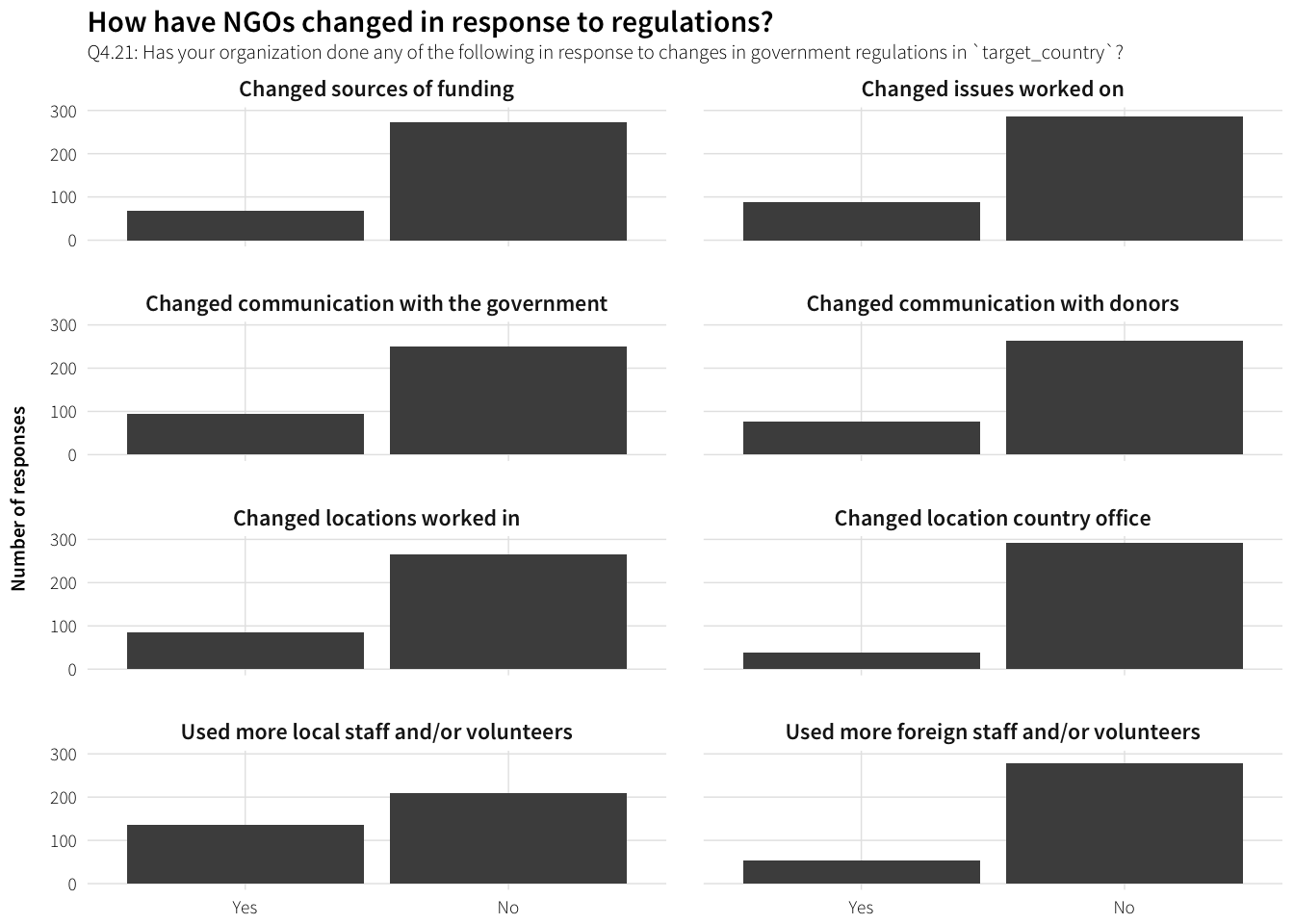#### Changed sources of funding

Q4.21a: Please describe how your organization changed its funding sources. What regulation were you responding to?

df.Q4.21.funding <- survey.countries.clean %>%
filter(!is.na(Q4.21_funding_TEXT)) %>%
arrange(Q4.21_funding_TEXT) %>% select(Q4.21_funding_TEXT)

datatable(df.Q4.21.funding)

#### Changed issues worked on

Q4.21b: Please describe how your organization changed which issues it works on. What regulation were you responding to?

df.Q4.21.issues <- survey.countries.clean %>%
filter(!is.na(Q4.21_issues_TEXT)) %>%
arrange(Q4.21_issues_TEXT) %>% select(Q4.21_issues_TEXT)

datatable(df.Q4.21.issues)

#### Changed communication with the government

Q4.21c: Please describe how your organization changed how it communicates with the government. What regulation were you responding to?

df.Q4.21.comm_govt <- survey.countries.clean %>%
filter(!is.na(Q4.21_comm_govt_TEXT)) %>%
arrange(Q4.21_comm_govt_TEXT) %>% select(Q4.21_comm_govt_TEXT)

datatable(df.Q4.21.comm_govt)

#### Changed communication with donors

Q4.21d: Please describe how your organization changed how it communicates with donors. What regulation were you responding to?

df.Q4.21.comm_donors <- survey.countries.clean %>%
filter(!is.na(Q4.21_comm_donors_TEXT)) %>%
arrange(Q4.21_comm_donors_TEXT) %>% select(Q4.21_comm_donors_TEXT)

datatable(df.Q4.21.comm_donors)

#### Changed locations worked in

Q4.21e: Please describe how your organization changed the locations it works in. What regulation were you responding to?

df.Q4.21.locations <- survey.countries.clean %>%
filter(!is.na(Q4.21_locations_TEXT)) %>%
arrange(Q4.21_locations_TEXT) %>% select(Q4.21_locations_TEXT)

datatable(df.Q4.21.locations)

#### Changed location country office

Q4.21f: Please describe how your organization changed the location of its country office. What regulation were you responding to?

df.Q4.21.country_office <- survey.countries.clean %>%
filter(!is.na(Q4.21_country_office_TEXT)) %>%
arrange(Q4.21_country_office_TEXT) %>% select(Q4.21_country_office_TEXT)

datatable(df.Q4.21.country_office)

#### Used more local staff and/or volunteers

Q4.21g: Please describe how your organization used more local staff or volunteers. What regulation were you responding to?

df.Q4.21.local_staff <- survey.countries.clean %>%
filter(!is.na(Q4.21_local_staff_TEXT)) %>%
arrange(Q4.21_local_staff_TEXT) %>% select(Q4.21_local_staff_TEXT)

datatable(df.Q4.21.local_staff)

#### Used more foreign staff and/or volunteers

Q4.21h: Please describe how your organization used more foreign staff or volunteers. What regulation were you responding to?

df.Q4.21.foreign_staff <- survey.countries.clean %>%
filter(!is.na(Q4.21_foreign_staff_TEXT)) %>%
arrange(Q4.21_foreign_staff_TEXT) %>% select(Q4.21_foreign_staff_TEXT)

datatable(df.Q4.21.foreign_staff)

### Have NGOs discussed regulations with government?

df.reg.discuss <- survey.countries.clean %>%
filter(!is.na(Q4.22)) %>%
group_by(Q4.22) %>%
summarise(num = n()) %>%
ungroup() %>%
mutate(Q4.22 = factor(Q4.22, levels=rev(levels(Q4.22)), ordered=TRUE))

plot.reg.discuss <- ggplot(df.reg.discuss, aes(x=num, y=Q4.22)) +
geom_barh(stat="identity") +
scale_x_continuous(expand=c(0, 0)) +
labs(x="Number of responses", y=NULL,
title="Have NGOs discussed regulations with government?",
subtitle="Q4.22: Has your organization discussed NGO regulations with government officials in target_country?") +
theme_ath()

plot.reg.discuss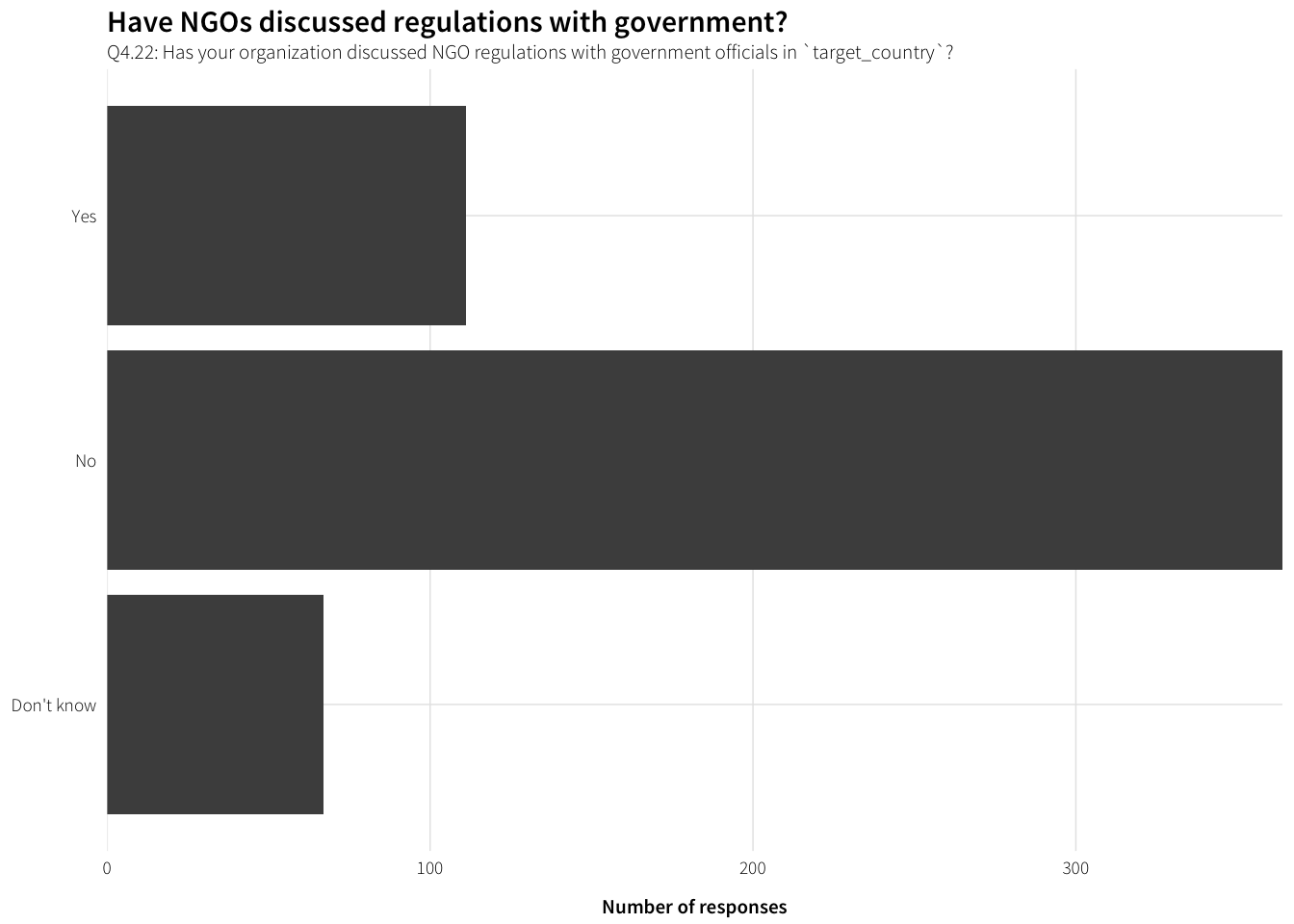### Have NGOs tried to change regulations?

df.reg.lobby <- survey.countries.clean %>%
filter(!is.na(Q4.23)) %>%
group_by(Q4.23) %>%
summarise(num = n()) %>%
ungroup() %>%
mutate(Q4.23 = factor(Q4.23, levels=rev(levels(Q4.23)), ordered=TRUE))

plot.reg.lobby <- ggplot(df.reg.lobby, aes(x=num, y=Q4.23)) +
geom_barh(stat="identity") +
scale_x_continuous(expand=c(0, 0)) +
labs(x="Number of responses", y=NULL,
title="Have NGOs tried to change regulations?",
subtitle="Q4.23: Has your organization tried to change NGO regulations in target_country?") +
theme_ath()

plot.reg.lobby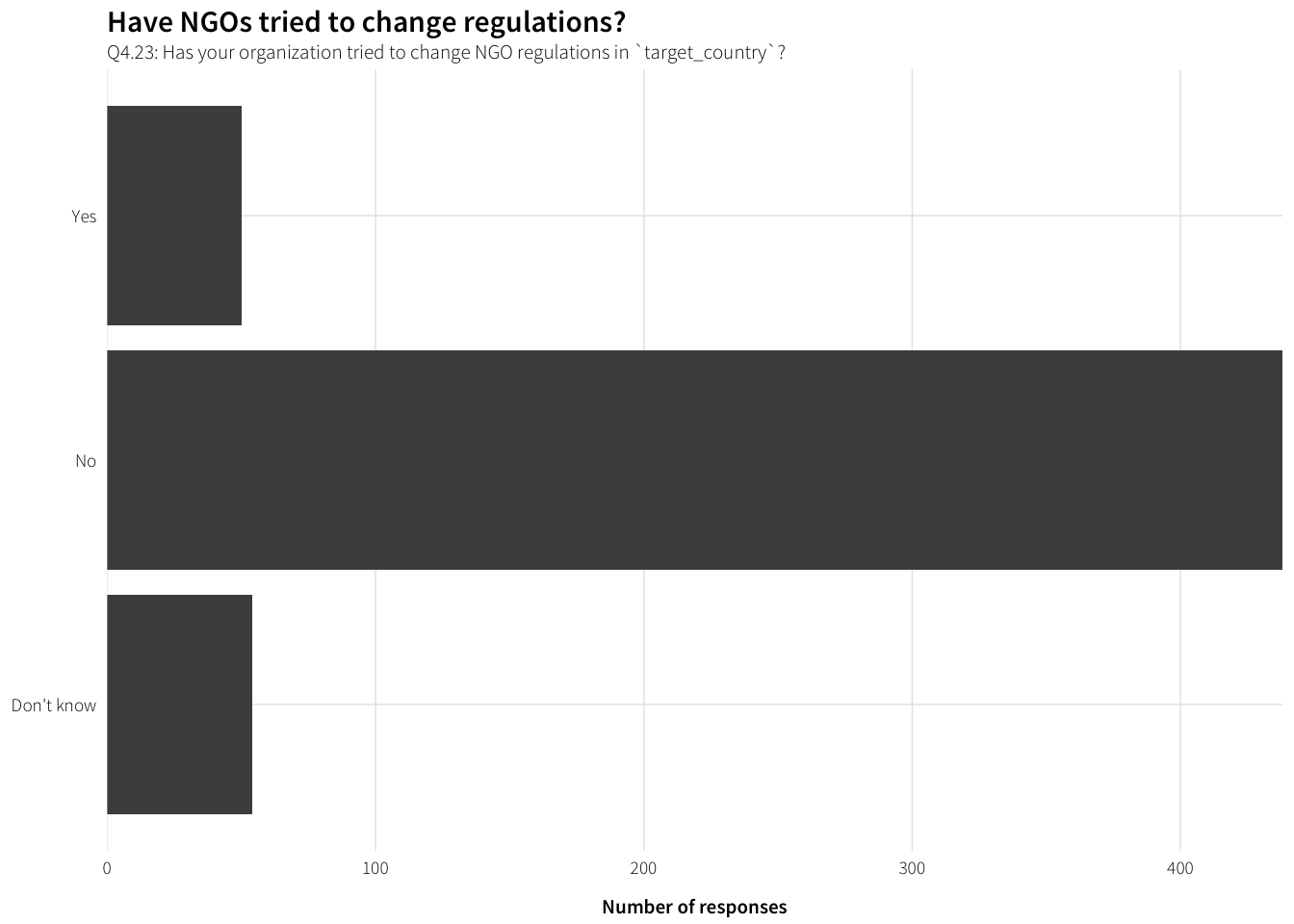## Final thoughts

df.Q5.1 <- survey.orgs.clean %>%
datatable(df.Q5.1)`